# Note 1

## 1 Introduction to Natural Language Processing

### What is natural language

• A discrete / symbolic / categorical system
• the word is a signifier that maps to a signified(idea or thing)
• the symbols of language can be encoded in several modalities: voice, gesture, writing, etc that are transmitted via continuous signals to the brain.

• Easy:
• Spell Checking
• Keyword Search
• Finding Synonyms
• Medium
• Parsing information from websites, documents, etc.
• Hard
• Machine Translation
• Semantic Analysis
• Co-reference

### How to represent words?

• Vectors.
• To perform well on most NLP tasks, we first need to have some notion of similarity and difference between words.
• Distance measures: Jaccard, Cosine, Euclidean, etc.

## 2 Word Vectors

• Want to encode word tokens each into some vector that represents a point in some sort of “word” space.
• There perhaps exists some N-dimensional space such that $N \ll Size(vocabulary)$ that is sufficient to encode all semantics of the language.
• Each dimension would encode some meaning that we transfer using speech.
• Eg. Semantic -> tense, count, gender.
• One-hot vector:
•  represent every word as $\mathbb{R}^{ V \cross 1}$ vector with all 0s and one 1.
• no notion of similarity, since dot product between vectors are all 0

## 3 SVD Based Methods

• Loop over corpus and accumulate word co-occurrence counts in form of a matrix $X$
• Then perform singular value decomposition on $X$ to get a $USV^T$ decomposition.
• Use rows of $U$ as word embeddings.

### 3.1 Word-Document Matrix

1. Assumption: words that are related will often appear in the same documents.
2.  $X$: word-document matrix(also known as document term matrix). $\mathbb{R}^{ V \cross M}$
3. It scales with the number of documents(M).

### 3.2 Window based Co-occurrence Matrix

1. Assumption: words that are related will appear close to each other in text
2.  $X$: word-word co-occurence matrix (count the number of times each word appears inside a window of of a particular size around the word of interest) $\mathbb{R}^{ V \cross V }$ X is an affinity matrix
3. It is fixed with vocabulary size, but window size is a hyper-parameter

### 3.3 Applying SVD to the co-occurrence matrix

• Observing the values on the diagonal entries in $S$ and cut off at some index $k$ based on the desired percentage variance captured.
• Select first $k$ columns of $U$ to be used as word vectors.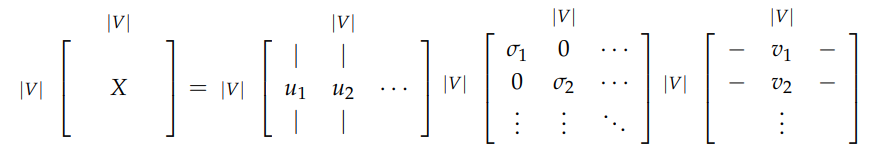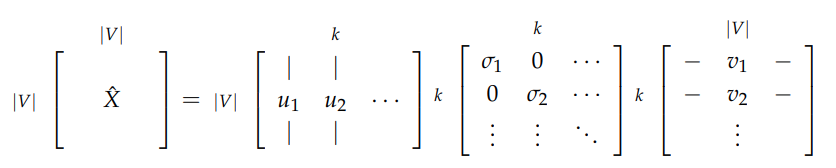• Both of these methods give us word vectors that are more than sufficient to encode semantic and syntactic (part of speech) information but are associated with many other problems:
1. Dimensions of matrix change very often. (new words, more documents)
2. Matrix is extremely sparse since most words do not co-occur
3. Matrix is very high dimensional $\approx 10 ^6\cross 10^6$
4. Quadratic cost to train (SVD)
5. Requires the incorporation of some hacks on X to account for the drastic imbalance in word frequency. (TFIDF / BM25)
• Some solutions to above problems:
1. Ignore function words such as “the”, “he”, “has”, etc
2. Apply a ramp window - weight co-occurrence count based on distance between the words in the document.
3. Use Pearson correlation and set negative counts to 0 instead of using just raw count.
• Iteration based methods solve many of these issues

## 4 Iteration Based Methods - Word2Vec

• Instead of computing and storing global information about some huge corpus, we try to create a model that is learned one iteration at a time and eventually be able to encode the probability of a word given its context.
• Context of a word is the set of $m$ surrounding words.
• Assumption: distributional similarity - similar words have similar context.
• Train a model whose parameters are the word vectors against a certain objective.
• Word2vec is a software package includes:
1. 2 algorithms: continuous bag-of-words(CBOW) and skip-gram.
• CBOW aims to predict a center word from the surrounding context
• Skip-gram predicts the distribution of context words from a center word
2. 2 training methods: negative sampling and hierarchical softmax
• Negative sampling defines an objective by sampling negative examples
• Hierarchical softmax defines an objective using an efficient tree structure to compute probabilities for all the vocabulary.

### 4.1 Language Models (Unigrams, Bigrams, etc)

• Create a model that assigns a probability to a sequence of tokens.
• Assumption: word occurrences are completely independent.
• Unigram model: $P(w_1, w_2, …, w_n) = \prod_{i=1}^{n}P(w_i)$
• Assumption: probability of the sequence depend on the pairwise probability of a word in the sequence and the word next to it.
•  Bigram model: $P(w_1,w_2,…,w_n) = \prod_{i=2}^{n}P(w_i w_{i-1})$

### 4.2 Continuous Bag of Words Model(CBOW)

• Treat {“The”, “cat”, “over”, “the”, “puddle”} as a context and from this words, be able to predict or generate the center word “jumped”.

• For each word, we try to learn two vectors, one to use when word is context word, another to use when word is center word.

•  $\mathit{V} \in \mathbb{R}^{n \cross V }$
•  $\mathit{U} \in \mathbb{R}^{ V \cross n}$
• How the model works:

1. Generate one-hot word vectors for the input context of size $m$: $(x^{c-m},…,x^{c-1},x^{c+1},…,x^{c+m})$
2. Get embedded word vectors for the context: $(v_{c-m}=Vx^{c-m},…,v_{c+m}=Vx^{c+m})$
3. Average these vectors to get $\hat{v} = \frac{v_{c-m}+v_{c-m+1}+…+v_{c+m}}{2m}$
4.  Generate a score vector $z=U\cdot\hat{v} \in \mathbb{R}^{ V }$ As the dot product of similar vectors is higher, it will push similar words close to each other(ideally predicted center word and true center word) in order to achieve a high score.
5.  Turn the scores into probabilities $\hat{y} \in \mathbb{R}^{ V }$ with softmax. Where $softmax(v) = \frac{e^{v_i}}{\Sigma_{k=1}^{ V }e^{y_k}} for \qquad i \in V$
6.  We want the predicted probabilities to match the true probabilities, $y\in\mathbb{R}^{ V }$ which is the one-hot encoded center word.
• Loss function:

•  Cross entropy $H(\hat{y}, y) = - \sum_{j=1}^{ V }y_jlog(\hat{y}_j)$
• It gives us a good measure of distance.
\begin{align} minimize J &=-logP(w_c|w_{c-m},...,w_{c+m}) \\ &= -logP(u_c|\hat{v}) \\ &= -log\frac{exp(u_c^T\hat{v})}{\Sigma_{j=1}^{|V|}exp(u_j^T\hat{v})} \\ &= -u_c^T\hat{v} + log\Sigma_{j=1}^{|V|}exp(u_j^T\hat{v}) \end{align}
• We optimize $U$ and $V$ via stochastic gradient descent (SGD).

• $U\leftarrow U-\alpha\nabla _uJ; V\leftarrow V-\alpha\nabla_vJ$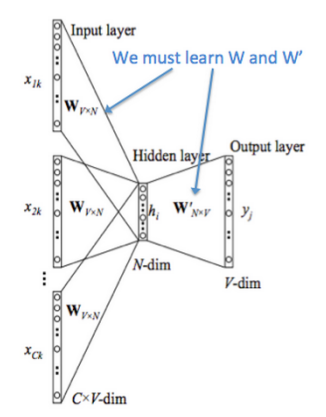### 4.3 Skip-Gram Model

• Given the center word “jump” the model will be asked to predict or generate the surrounding words.

• Essentially, we are swapping $x$ and $y$ in the model.

• Steps:

1.  Generate input one hot vector $x\in\mathbb{R}^{ V }$ for the center word.
2. Look up the embedding vector $v_c=V_x\in\mathbb{R}^n$
3.  Generate a score vector $z=Uv_c\in \mathbb{R}^{ V }$
4. Turn the score vector into probabilities $\hat{y}$ with softmax
5. Desire the output with target $y^{c-m},…,y^{c+m}$
6. We invoke a Naive Bayes assumption(conditional independence) assuming all output words are completely independent.
\begin{align} minimize J &=-logP(w_{c-m}, ..., w_{c+m}|w_c) \\ &= -log\prod_{j=0,j\neq m}^{2m}P(w_{c-m+j}|w_c) \\ &= -log\prod_{j=0,j\neq m}^{2m}P(u_{c-m+j}|v_c) \\ &= -log\prod_{j=0,j\neq m}^{2m}\frac{exp(u_{c-m+j}^Tv_c)}{\sum_{k=1}^{|V|}exp(u_{k}^Tv_c)} \\ &= -\sum_{j=0,j\neq m}^{2m}u_{c-m+k}^Tv_c+2mlog\sum_{k=1}^{|V|}exp(u_k^Tv_c) \end{align} $J=-\sum_{j=0,j\neq m}^{2m}logP(u_{c-m+j}|v_c) = \sum_{j=0,j\neq m}^{2m}H(\hat{y}, y_{c-m+j})$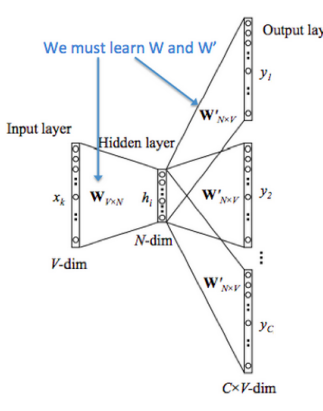### 4.4 Negative Sampling

•  One problem with the objective function is that it is a summation over $V$.
• One solution is to approximate it.

• For each training step, we sample several negative examples from a noise distribution $P_n(w)$ whose probabilities match the ordering of the frequency of the vocabulary.

• Considering a pair of word and context $(w, c)$ let:

•  $P(D=1 w,c)$ be the probability that the pair came from the data.
•  $P(D=0 w,c)$ be the probability that the pair did not came from the data.
•  Model $P(D=1 w,c) = \sigma(v_c^Tv_w) = \frac{1}{1+ e^(-v_c^Tv_w)}$
• MLE approach to maximize these two probabilities:

\begin{align} \theta &= \underset{\theta}{argmax} \prod_{(w,c)\in D} P(D=1|w,c,\theta)\prod_{(w,c)\in \tilde{D}} P(D=0|w,c,\theta) \\ &= \underset{\theta}{argmax} \prod_{(w,c)\in D} P(D=1|w,c,\theta) \prod_{(w,c)\in \tilde{D}}(1-P(D=1|w,c,\theta)) \\ &= \underset{\theta}{argmax} \sum_{(w,c)\in D}logP(D=1|w,c,\theta) + \sum_{(w,c)\in\tilde{D}}log(1-P(D=1|w, c,\theta)) \\ &= \underset{\theta}{argmax} \sum_{(w,c)\in D}log\frac{1}{1+exp(-u_w^Tv_c)} + \sum_{(w,c)\in\tilde{D}}log(1-\frac{1}{1+exp(-u_w^Tv_c)}) \\ &= \underset{\theta}{argmax} \sum_{(w,c)\in D}log\frac{1}{1+exp(-u_w^Tv_c)} + \sum_{(w,c)\in\tilde{D}}log(\frac{1}{1+exp(u_w^Tv_c)}) \\ \end{align}
• Maximizing the likelihood is the same as minimizing the negative log likelihood.
$J=-\sum_{(w,c)\in D}log\frac{1}{1+exp(-u_w^Tv_c)} - \sum_{(w,c)\in\tilde{D}}log(\frac{1}{1+exp(u_w^Tv_c)})$
• $\tilde{D}$ is false or negative corpus, we can generate on the fly by randomly sampling from the word bank.
• Now with negative sampling the skip-gram model’s objective function becomes:
$-log\sigma(u_{c-m+j}^T\cdot v_c)-\sum_{k=1}^{K}log\sigma(-\tilde{u}_k^T\cdot v_c)$
• For CBOW, the objective function will be:
$-log\sigma(u_c^T\cdot\hat{v}) - \sum_{k=1}^{K}log\sigma(-\tilde{u}_k^T\cdot \hat{v})$
• For $P_n(w)$, what seems to work best is the Unigram Model raised to the power of 3/4.

### 4.5 Hierarchical Softmax

• Hierarchical softmax is an efficient alternative to the normal softmax.
• In practice, hierarchical softmax tends to be better for infrequent words, while negative sampling works better for frequent words and lower dimensional vectors.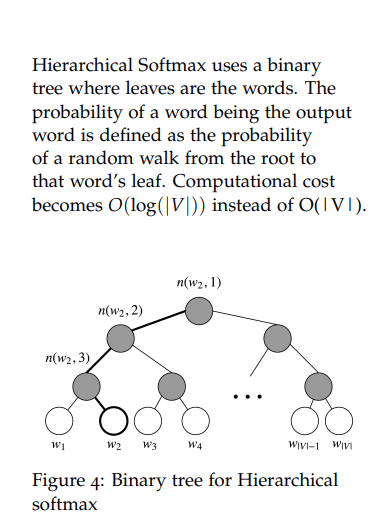• Hierarchical softmax uses a binary tree to represent all words in the vocabulary. Each leaf is a a word, and there is a unique path from root to leaf.
•  There is no output representation for words. Instead each node of the graph is associated to a vector that the model is going to learn. The probability of a word $w$ given a vector $w_i$, $p(w w_i)$ is equal to the probability of a random walk starting in the root and ending in the lead node corresponding to $w$.
•  Cost to compute the probability is $O(log( V ))$
$P(w|w_i)=\prod_{j=1}^{L(w)-1}\sigma([n(w, j+1)=ch(n(w,j))]\cdot v_{n(w,j)}^Tv_{w_i}) \\ where \\ [x] = \begin{cases} 1 & \text{ if x is true}\\ -1 & \text{otherwise} \end{cases}$
• $n(w, i)$ is the root $n(w, L(w))$ is the parent node of $w$
• $ch(n)$ indicate the left children of node $n$
• $[n(w, j+1)=ch(n(w,j))]$ will be 1 if node is left -1 if node is right, thus ensures proper normalization.
• To train the model, our goal is to minimize the negative log likelihood by updating vectors of the nodes in the binary tree.

# Note 2

## 1 Global Vectors for Word Representation (GloVe)

### 1.1 Comparison with Previous Methods

1. Count based
• Rely on Matrix factorization. (LSA, HAL)
• Effectively leverage global statistical information
• Ok with word similarities, but poorly on other tasks -> vector space structure is sub-optimal.
2. Shallow window-based
• Skip-gram and CBOW
• Learn embedding by making predictions in local context windows
• Capture complex linguistic patterns beyond word similarity
• Fail to make use of the global co-occurrence statistics
3. GolVe
• Using global statistics to predict the probability of word pair co-occurrence

### 1.2 Co-occurrence Matrix

• $X_{ij}$ indicates the number of time word $j$ occur in the context of word $i$
• $X_i=\sum_kX_{ik}$ be the number of times any word $k$ appears in the context of word $i$
•  $P_{ij} = p(w_j w_i) = \frac{X_{ij}}{X_{i}}$ is the probability of $j$ appearing in the context of word $i$

### 1.3 Least Squares Objective

• softmax compute the probability of word $j$ appears in the context of word $i$ $$Q_{ij} = \frac{exp(u_j^Tv_i)}{\sum_{w=1}^{W}exp(u_w^Tv_i)}$$

• Global corss-entropy objective function trained in an on-line, stochastic fashion $$J = -\sum_{i \in \text{corpus}}\sum_{j \in \text{context(i)}}logQ_{ij}$$

• It is more efficient to group together the same values for $i$ and $j$ $$J=-\sum_{i=1}^{W}\sum_{j=1}^{W}X_{ij}logQ_{ij}$$ where the value of co-occurring frequency is given by the co-occurrence matrix X

• One drawback of the cross-entropy loss is that it requires the distribution $Q$ to be properly normalized, which involves the expensive summation over the entire vocabulary.

• Instead, we use a least square objective in which the normalization factors in $P$ and $Q$ are discarded: $$\hat{J}=\sum_{i=1}^{W}\sum_{j=1}^{W}X_{ij}(\hat{P_{ij}}-\hat{Q_{ij}})^2$$ where $\hat{P_{ij}} = X_{ij}$ and $\hat{Q_{ij}} = exp(u_j^Tv_i)$ are the unnormalized distributions.

• New problem: $X_{ij}$ often takes on very large values and makes the optimization difficult.

• Solution: minimize the squared error of the logarithm of $\hat{P}$ and $\hat{Q}$ \begin{align} \hat{J} &= \sum_{i=1}^{W} \sum_{j=1}^{W}X_i(log(\hat{P_{ij}}) - log(\hat{Q_{ij}}))^2\\ &=\sum_{i=1}^{W} \sum_{j=1}^{W}X_i(u_j^Tv_i - logX_{ij})^2 \\ &=\sum_{i=1}^{W} \sum_{j=1}^{W}f(X_{ij})(u_j^Tv_i - logX_{ij})^2 \end{align} where $f(X_{ij})$ is a more general weighting function than the raw $X_i$

## 2 Evaluation of Word Vectors

### 2.1 Intrinsic Evaluation

• Evaluate word vectors on specific intermediate subtasks. Like analogy completion for question answering. Reason? If evaluated with the final downstream task, the entire pipeline will be retrained from scratch each time we need to tweak the word vector learning.
• Requirement: the intrinsic evaluation has a positive correlation with the final task performance.

### 2.2 Extrinsic Evaluation

• Evaluate word vectors on the real task.
• Typically, optimizing over an under performing extrinsic evaluation system does not allow us to determine which specific subsystem is at fault and this motivates the need for intrinsic evaluation.

### 2.3 Intrinsic Evaluation Example: Word Vector Analogies

• $a:b::c:?$

• find $d$ such that it maximizes the cosine similarity $$d=\underset{i}{argmax}\frac{(x_b-x_a+x_c)^Tx_i}{||x_b-x_a+x_c||}$$

• semantci: capital city -> country

• syntactic: adj -> it’s superlative form

### 2.4 Analogy Evaluations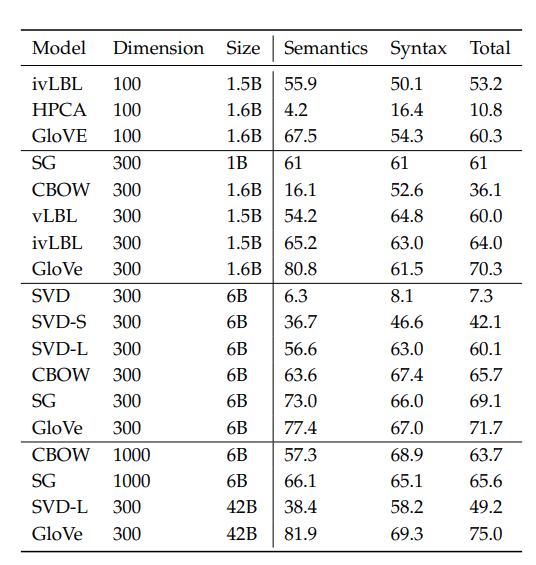• Performance is heavily dependent on the model used for word embedding.
• Performance increases with larger corpus sizes
• Performance is lower for extremely low dimensional word vectors
• Extremely high dimensional vectors with CBOW/SG is reasonably robust to noise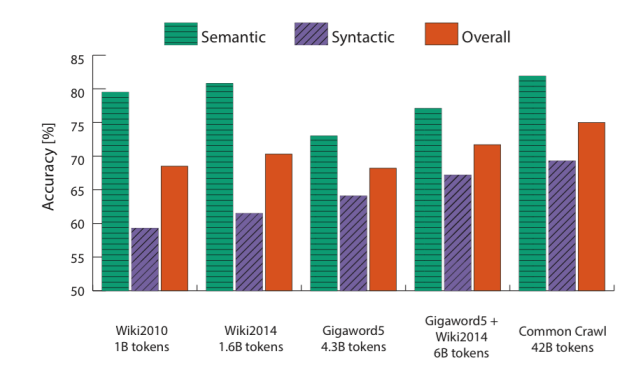### 2.5 Correlation Evaluation

• Another simple way to evaluate the quality of word vectors is by asking humans to assess the similarity between two words on a fixed scale and then comparing this with the cosine similairity between the corresponding word vectors.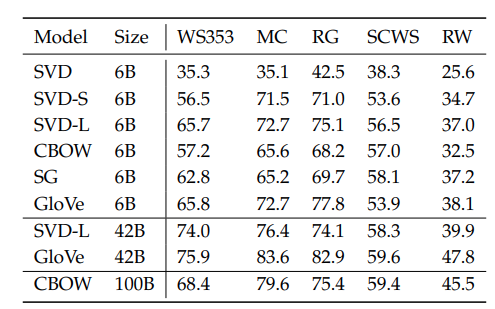### 2.6 Dealing With Ambiguity

• Improving word representations via global context and multiple word prototypes. Huang et.al. 2012

## 3. Training for Extrinsic Tasks

### 3.1 Problem Formulation

• Sentiment analysis: given a text, classify it to be negative or positive.
• Named-entity recognition(NER): given a context and a center word, classify the center word to be one of many classes.

### 3.2 Retraining Word Vectors

• If we retrain word vectors using the extrinsic task, we need to ensure that the training set is large enough to cover most words from the vocabulary.
• When retrain word vectors over a small set of the vocabulary, these words are shifted in the word space and as a result, the performance over the final task could actually reduce.

### 3.3 Softmax Classification and Regularization

• Softmax classification: $$p(y_j=1|x) = \frac{exp(W_j\cdot x)}{\sum_{c=1}^{C}exp(W_c\cdot x)}$$

• Using the cross-entropy loss: $$-\sum_{j=1}^{C}y_jlog(p(y_j=1|x)) = -\sum_{j=1}^{C}y_j log(\frac{exp(W_j\cdot x)}{\sum_{c=1}^{C}exp(W_c\cdot x)})$$

• Since there is only one correct answer, let $k$ be the correct one. The loss function simplify to: $$-log(\frac{exp(W_k\cdot x)}{\sum_{c=1}^{C}exp(W_c\cdot x)})$$

• Extend to a dataset of N points: $$-\sum_{i=1}^{N}log(\frac{exp(W_{k(i)}\cdot x^{(i)})}{\sum_{c=1}^{C}exp(W_c\cdot x^{(i)})})$$

• We have $C\cdot d+|V|\cdot d$ parameters to update each time. To avoid, over fitting, we can pose the Bayesian belief that parameters $(\theta)$ should be small in magnitude: $$-\sum_{i=1}^{N}log(\frac{exp(W_{k(i)}\cdot x^{(i)})}{\sum_{c=1}^{C}exp(W_c\cdot x^{(i)})}) + \lambda \sum_{k=1}^{C\cdot d + |V|\cdot d}{\theta_k}^2$$

### 3.4 Window Classification

• Instead of using just the word of interest, use a sequence that is a center word preceded and suceeded by context words.
• Generally, narrower window sizes lead to better performance in syntactic tests while wider windows lead to better performance in semantic tests.

### 3.5 Non-linear Classifiers

• Non-linear decision boundaries to solve not linear separable classification problem.

# Note 3

## 1 Dependency Grammar and Dependency Structure

• Parse trees in NLP are used to analyze the syntactic structure of sentences.
1. Constituency structures - phrase structure grammar to organize words into nested constituents.
2. Dependency structures - shows which words depend on (modify or are argument of) which other words.
• Dependency tree for the sentence: “Bills on ports and immigration were submitted by Senator Brownback, Republican of Kansas.”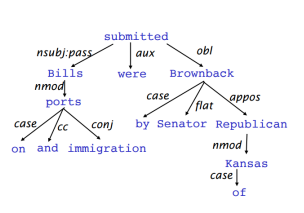### 1.1 Dependency Parsing

• Task of analyzing the syntactic dependency structure of a given input sentence S.
• Output is a dependency tree/graph $G$ where the words are connected by typed dependency relations.
1. Learning: Given a training set $D$ of sentences annotated with dependency graphs, induce a parsing model M that can be used to parse new sentences.
2. Parsing: Given a parsing model M and a sentence S, derive the optimal dependency graph D for S according to M.

### 1.2 Transition-Based Dependency Parsing

• State machine which defines the possible transitions to create the mapping from the input sentence to the dependency tree.
• Learning: induce a model to predict the next transition
• Parsing: construct the optimal sequence of transitions
• Most transition-based systems do not make use of a formal grammar.

### 1.3 Greedy Deterministic Transition-Based Parsing

• Nivra 2003, showed to be better in both performance and efficiency than traditional feature-based discriminative dependency parsers.

• States:
• For any sentence $S=w_0w_1…w_n$ a state can be described with a triple $c=(\sigma, \beta,A)$
1. a stack $\sigma$ of words $w_i$ from $S$
2. a buffer $\beta$ of words $w_i$ from $S$
3. a set of dependency arcs $A$ of the form $(w_i, r, w_j)$, where $w_i, w_j$ are from $S$, and $r$ describes a dependency relation.
• It follows that for any sentence $S=w_0w_1…w_n$,
1.  an initial state $c_0$ is of the form $( w_0 {\sigma}, [w_1,…,w_n]{\beta}, \emptyset)$
2. a terminal state has the form $({\sigma}, []{\beta}, A)$
• Transitions
• SHIFT: remove the first word in the buffer and push it on top of the stack
• LEFT-ARC: add a dependency arc $(w_j, r, w_i)$ to the arc set $A$, where $w_i$ is the word second to the top of the stack and $w_j$ is the word at the top of the stack. Remove $w_i$ from the stack
• RIGHT-ARC: dd a dependency arc $(w_i, r, w_j)$ to the arc set A, where $w_i$ is the word second to the top of the stack and $w_j$ is the word at the top of the stack. Remove $w_j$ from the stack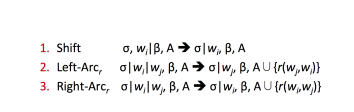### 1.4 Neural Dependency Parsing

• Feature Selection:
• $S_{word}$ vector representations for some of the words in $S$ (and their dependents) at the top of the stack $\sigma$ and buffer $\beta$
• $S_{tag}$: Part-of-Speech (POS) tags for some of the words in $S$. POS tags comprise a small, discrete set: $P={NN,NNP,NNS,DT,JJ,…}$
• $S_{label}$: The arc-labels for some of the words in $S$. The arc-labels comprise a small discrete set, describing the dependency relation: $L={amod, tmod,nsubj,csubj,dobj,…}$
• For each feature type, we will have a corresponding embedding matrix, mapping from the feature’s one hot encoding , to a $d-$dimensional dense vector representation.
• For example:
• $S_{word}$: top 3 words on stack and buffer, the first and second leftmost / right most children of the top two words on the stack. the leftmost of leftmost / rightmost of rightmost children of the top two words on the stack. 18 elements in total
• $S_{tag}$: 18 corresponding tags
• $S_{label}$: 12 in total.
• Model: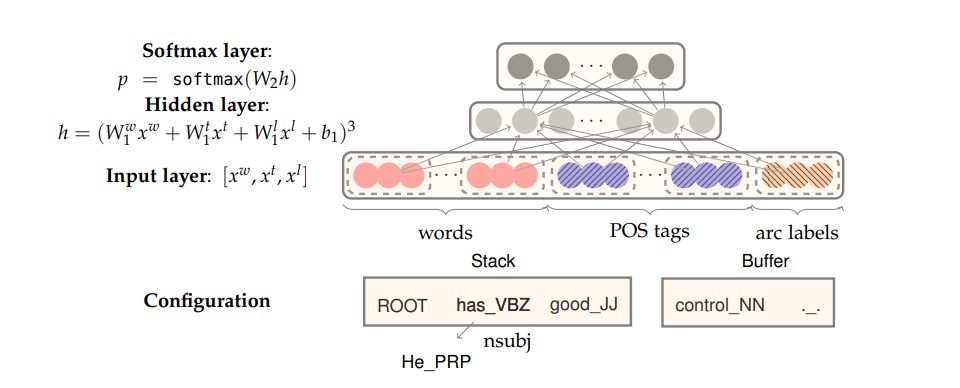# Note 4

## 1 Language Models

### 1.1 Introduction

• Language models compute the probability of occurrence of a number of words in a particular sequence.

• The probability of a sequence of $m$ words ${w_1, …, w_m}$ is $P(w_1,…,w_m)$

• It is usually conditioned on a window of $n$ previous words rather than all previous words: $$P(w_1, ..., w_m) = \prod_{i=1}^{m}P(w_i|w_1,...,w_{i-1}) \approx \prod_{i=1}^{m}P(w_i|w_{i-n},...,w_{i-1})$$

### 1.2 n-gram Language Models

• One way to compute the above probabilities is n-gram.

• Bigram: $$p(w_w|w_1) = \frac{count(w_1, w_2)}{count(w_1)}$$

• Trigram: $$p(w_3|w_1,w_2) = \frac{count(w_1, w_2, w_3)}{count(w_1, w_2)}$$

• Main issues with n-gram Language Models:

1. Sparsity problems:
• If $w_1, w_2$ never appear together, the numerator for trigram is 0. Add smoothing a small $\delta$.
• If $w_1, w_2$ never appear together, the denominator for trigram is 0. Can condition on $w_2$ alone, called backoff.
• Increasing $n$ makes sparsity problems worse. Typically, $n\leq 5$
2. Storage problems with n-gram Language Models:
• Store counts for all n-grams is large and it grow fast as corpus size grows.

### 1.3 Window-based Neural Language Model

• Bengio et al Neural Probabilistic Language Model, first large-scale deep learning for natural language processing model.
• It learns a distributed representation of words, along with the probability function for word sequences expressed in terms of these representations.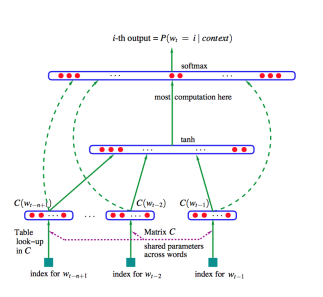• Consider window-based model as Language Model conditioned on fixed size history of information.

## 2 Recurrent Neural Networks (RNN)

• RNN can conditioning the Language Model on all previous words.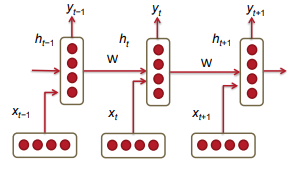$$h_t = \sigma(W^{(hh)}h_{t-1}+W^{(hx)}x_{[t]}) \\ \hat{y}_t = softmax(W^{(S)}h_t)$$

• $W^{(hh)}$ and $W^{(hx)}$ are shared across time, applied repeatedly at each step.
• Thus model learn fewer parameters
• And is independent of the length of the input sequence
• Defeating the curse of dimensionality

### 2.1 RNN Loss and Perplexity

• RNN trained with cross entropy loss
• At a given time $t$ the loss is
$J^{(t)}(\theta) = -\sum_{j=1}^{|V|}y_{t,j}\cross log(\hat{y}_{t,j})$
• Aggregate over the corpus $$J = \frac{1}{T}\sum_{t=1}^{T}J^{(t)}(\theta) = -\frac{1}{T}\sum_{t=1}^{T}\sum_{j=1}^{|V|}y_{t.j}\cross log(\hat{y}_{t, j})The$$

• The perplexity is defined as: $2^{J}$

Lower value imply more confident in predicting the next word in sequence

1. process input sequences of any length
2. model size independent of sequence lengths
3. computation for step $t$ can (in theory) use information from many steps back
1. computation is slow - because it is sequential, can’t be parallelized
2. in practice, it is difficult to access information from many steps back due to vanishing and exploding gradients.
• RNNs can be used for many tasks, such as tagging (POS, NER), sentence classification(category, sentiment), and as encoder or decoder modules.

\begin{align} \frac{\partial E}{\partial W} &= \sum_{t=1}^{T}\frac{\partial E_t}{\partial W} \\ \frac{\partial E_t}{\partial W} &= \sum_{k=1}^{t}\frac{\partial E_t}{\partial y_t}\frac{\partial y_t}{\partial h_t}\frac{\partial h_t}{\partial h_k}\frac{\partial h_k}{\partial W} \\ \frac{\partial h_t}{\partial h_k} &= \prod_{j=k+1}^{t}\frac{\partial h_{j}}{\partial h_{j-1}}=\prod_{j=k+1}^{t}W^T\cross diag[f'(j_j-1)] \\ \frac{\partial E}{\partial W} &= \sum_{t=1}^{T}\sum_{k=1}^{t}\frac{\partial E_t}{\partial y_t}\frac{\partial y_t}{\partial h_t}(\prod_{j=k+1}^{t}\frac{\partial h_j}{\partial h_{j-1}})\frac{\partial h_k}{\partial W} \end{align}

$\partial h_{j} / \partial h_{j-1}$ is the Jacobian matrix for h. $$\|\frac{\partial h_j}{\partial h_{j-1}}\| \leq \|W^T\| \|diag[f'(h_{j-1})] \| \leq \beta_W \beta_h$$ Where $\beta_W$ and $\beta_h$ are the upper bound values for the norms of the two matrices. $f’(h_{j-1})$ can only be as large as 1, given sigmoid activation. $$\|\frac{\partial h_t}{\partial h_k}\| = \| \prod_{\partial h_{j-1}}^{\partial h_{j}} \| \leq (\beta_W\beta_h)^{t-k}$$

• if $\beta_W\beta_h$ get smaller or larger than 1, with $t-k$ sufficiently large, the gradients blows or diminishes very quickly.
• Gradient Explosion, i.e. gradient overflow the floating number limits becomes NaN, can be detected.
• Gradient Vanish, i.e. gradient becomes 0, can be undetected. It will be hard to tell whether it is that:
• No relationship last that long, or
• We can’t capture this due to gradient vanishing.

### 2.4 Solution to the Exploding & Vanishing Gradients

1. For exploding gradient: gradient clipping $$\hat{g} \leftarrow \frac{\partial E}{\partial W} \\ if \quad \|\hat{g}\| \geq threshold \quad then \\ \hat{g} \leftarrow \frac{threshold}{\|\hat{g}\|}\hat{g}$$

1. identity matrix initialization
2. use ReLU activations

### 2.5 Deep Bidirectional RNNs

1. Bidirectional \begin{align} \overrightarrow{h_t} &= f(\overrightarrow{w_{x_t}} + \overrightarrow{V} \, \, \, \overrightarrow{h}_{t-1} + \overrightarrow{b}) \\ \overleftarrow{h_t} &= f(\overleftarrow{w_{x_t}} + \overleftarrow{V} \, \, \, \overleftarrow{h}_{t-1} + \overleftarrow{b}) \\ \hat{y}_t &= g(Uh_t + c) = g(U[\overrightarrow{h}_t; \overleftarrow(h)_t] + c) \end{align}

2. Stacked RNN $$\overrightarrow{h}^{(i)}_t = f(\overrightarrow{W}^{(i)} h^{(t-1)} + \overrightarrow{V}^{(i)} \overrightarrow{h}^{(i)}_{t-1} + \overrightarrow{b}^{(i)})$$

3. The two can be combined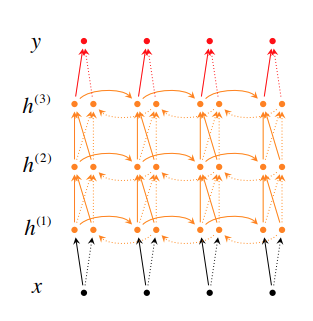### 2.6 Application: RNN Translation Model

• Encoder-Decoder RNN for translation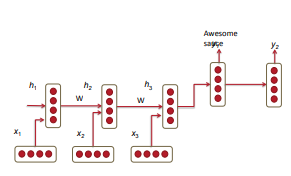1. Encoder RNN process the input sequence and produce a final hidden state, that is feed into decoder RNN as the initial hidden state.
2. The decoder RNN gets a <BOS> token as initial input and treat each of the previous prediction as input to the current step.
• Extensions:

• Use dedicated Encoder and Decoder

• Use Bidirectional RNN

• Use Stacked RNN

• Compute every hidden state in the decoder using three inputs

1. Previous hidden

2. Previous step prediction

3. Last hidden from encoder

• Sometimes worth trying instead of $ABC\rightarrow XY$, do $CBA\rightarrow XY$

## 3. Gated Recurrent Units

• Although RNNs can theoretically capture long-term dependencies, they are very hard to actually train to do this.

• Gated recurrent unites are designed in a manner to have more persistent memory thereby making it easier for RNNs to capture long-term dependencies. \begin{align} \text{update gate} \qquad z_t &= \sigma(W^{(z)}x_t + U^{(z)}h_{t-1}) \\ \text{reset gate} \qquad r_t &= \sigma(W^{(r)}x_t + U^{(r)}h_{t-1}) \\ \text{new memory} \qquad \tilde{h}_t &= tanh(r_t\circ Uh_{t-1} + Wx_t) \\ \text{hidden state} \qquad h_t &= (1-z_t)\circ\tilde{h}_t+z_t\circ h_{t-1} \\ \end{align}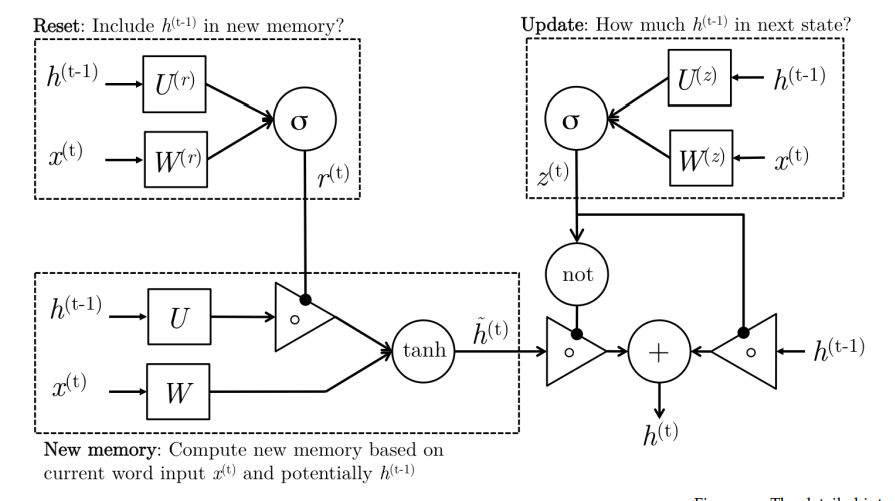## 4. Long-Short-Term-Memories

• The motivation for LSTM is similar to GRU, the architecture of unit is a bit different.

• LSTM has a separate cell state that is kept hidden. Hidden state is just a exposure of that cell state. \begin{align} \text{input gate} \qquad i_t &= \sigma(W^{(i)}x_t + U^{(i)}h_{t-1}) \\ \text{forget gate} \qquad f_t &= \sigma(W^{(f)}x_t + U^{(f)}h_{t-1}) \\ \text{output gate} \qquad o_t &= \sigma(W^{(o)}x_t + U^{(o)}h_{t-1}) \\ \text{new memeory cell} \qquad \tilde{c}_t &= tanh(W^{(c)}x_t + U^{(c)}h_{t-1}) \\ \text{final state cell} \qquad c_t &= f_t \circ c_{t-1} + i_t\circ \tilde{c}_t \\ h_t &= o_t \circ tanh(c_t) \\ \end{align}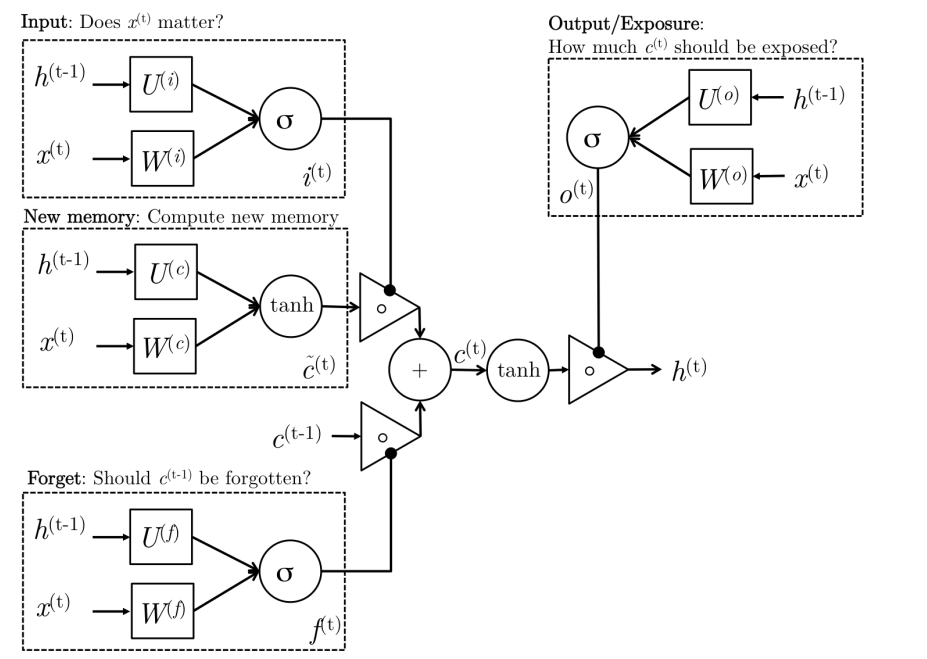# Note 5 Machine Translation

## 0 Statistical Machine Translation

•  probabilistic model and bayes rule: $argmax_yP(y x) = argmax_yP(x y)P(y)$
• where $P(y)$ is the language model learned from monolingual data.
•  and $P(x y)$ is the translation model learned from parallel data.
• Alignment - word-level correspondence between source sentence x and target sentence y
•  decompose $P(x y)$ to $P(x, a y)$
• alignments $a$ are latent variables learned with EM algorithm.
• Dynamic programming is used for globally optimal solutions (Viterbi algorithm)

## 1 Neural Machine Translation with Seq2Seq

• Type of Seq2Seq usage:
1. Translation
2. Conversation
3. Summarization

### 1.1 Brief Note on Historical Approaches

• Before neural network approach, translation systems were based on probabilistic models constructed from:
1. a translation model, telling us what a sentence / phrase in a source language most likely translates into
2. a language model, telling us how likely a given sentence / phrase is overall
• Phrase-based systems were most common prior to Seq2Seq. It is difficult to handle long-term dependencies with phrase-based system.

### 1.2 Sequence-to-sequence Basics

• Appeared in 2014 for English-French translation

• It is an end-to-end model made up of two RNNs:

• an encoder, which takes the model’s input sequence and encodes it into a fixed-size “context vector”
• an decoder, which uses the context vector from encoder as a “seed” from which to generate an output sequence.

### 1.3 Seq2Seq architecture - encoder

• Use a encoder RNN to process the input token sequence and the final hidden state will become the context vector.
• Usually the Seq2Seq encoders will process the input sequence in reverse. It is done under the reasoning that the last thing the encoder sees will (roughly) corresponds to the first thing that the model ouputs. this makes it easier for the decoder to “get started”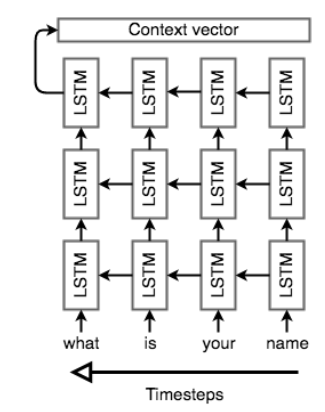### 1.4 Seq2Seq architecture - decoder

• Use a decoder RNN to generate output sequence based of the words it generated so far, as well as the context.
• The decoding process is kicked off with a special token <EOS> (if use the same RNN for both encoding and decoding) or <BOS> (If use dedicated networks)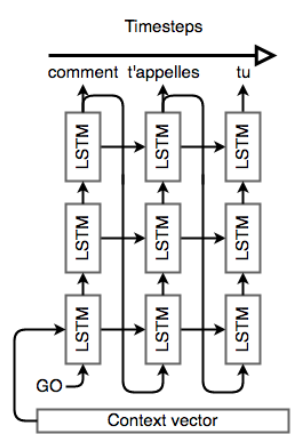### 1.5 Bidirectional RNNs

• Use bidirectional RNNs to traversing a sequence in both directions and concatenating the resulting final hidden states and cell states.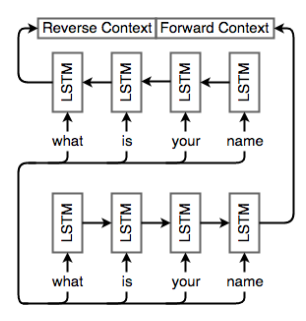## 2 Attention Mechanism

### 2.1 Motivation

• Bahdanau et al. 2016 proposing attention to address the issue with a single “context vector” for sequence-to-sequence models.
1. Different parts of an input have different levels of significance
2. Different parts of the output may consider different parts of the input “important”
• Attention mechanisms make use of this observation by providing the decoder network with a chance to look at the entire input sequence at every decoding step. The decoder can then decide what input words are important at any point in time.

### 2.2 Bahdanau et al. NMT model

1. Encoder: Use RNN to process input sequence and collect $(h_1,…,h_n)$

2. Decoder: $s_i=f(s_{i-1}, y_{i-1}, c_i)$ Where $s_{i-1}$ is the previous hidden, $y_{i-1}$ is the previous prediction, $c_i$ is the context from the input sequence that is relevant for the current decoding job. It is calculated as follow \begin{align} e_{i,j} &= a(s_{i-1}, h_j) \\ \alpha_{i,j} &= \frac{exp(e_{i,j})}{\sum_{k=1}^{n}exp(e_{i,k})} \\ c_i &=\sum_{j=1}^{n} \alpha_{i,j}h_j \\ \end{align} That is:

1. We use the hidden state $s_{i-1}$ to query the encoder hidden states, and create a score for each.
2. Use softmax to convert these scores into a proper probability
3. Finally compute the context vector as weighted average of the encoder hidden states.
• Ideally the softmax will put higher value on important stuff.

### 2.3 Connection with translation alignment

• The attention-based model learns to assign significance to different parts of the input for each step of the ouput.
• In the context of translation, attention can be thought of as “alignment”
• Bahdanau et al. argue that the attention scores $\alpha_{i,j}$ at decoding step $i$ signify the words in the input sequence that align with the word $i$ in the output sequence.

### 2.4 Performance on long sentence

• As the sequence length grows, models do not use attention will miss information.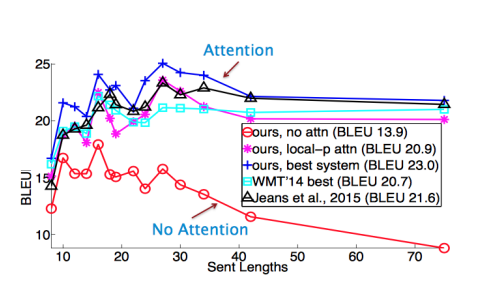### 2.5 Global / Local Attention

• Many ways to do attention. Luong et al’s attention are using current hidden state to do attention and modify what to output at the current step. Sort of post activation attention, compare to Bahdanau’s pre activation attention.
• And they added some control after attention about how much around the aligned position should the information be aggregated as context.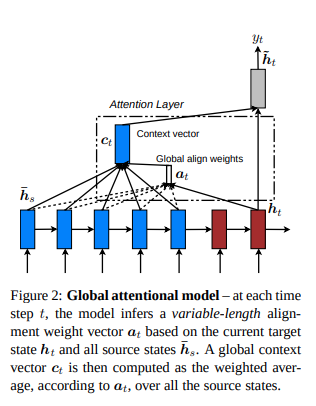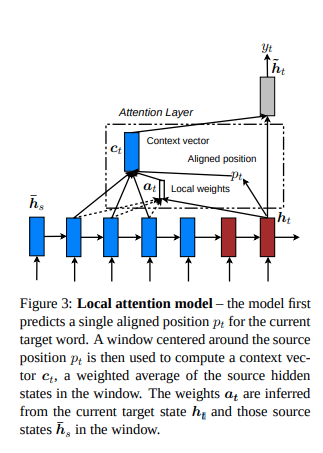1. A Seq2Seq model that accepts as input a sequence of words and a token specifying what language to translate into.
2. The model uses shared parameters to translate into any target language.
3. It enabled “zero-short translation”, in which we can translate between two languages for which we have no translation training data. They can actually generate reasonable Japanese-Korean translations where they only had Japanese-English and Korean-English in training data.
4. This is showing: the decoding process is not language-specific, the model is in fact maintaining an internal representation of the input / output sentences independent of the actual languages involved.

## 4 Sequence model decoders

• Consider a model that computes the probability $P(\bar{s}|s)$ of a translation $\bar{s}$ given the original sequence $s$. We want to pick the translation $\bar{s}*$ that has the best probability. $$\bar{s}*=argmax_{\bar{s}}(P(\bar{s}|s))$$

•  As the search space can be huge$V ^{len(s)}$, we need to shrink its size:
1. Exhaustive search: compute the probability of every possible sequence, and chose the one with the highest probability. Too complex, NP_complete.

2. Ancestral sampling: at time step $t$, we sample $x_t$ based on the conditional probability of the word at step $t$ given the past. $$x_t \sim P(x_t|x_1, \dots, x_n)$$ High variance

3. Greedy search: at time step $t$, we pick the most probable token. $$x_t=argmax_{\tilde{x_t}}P(\tilde{x_t}|x1,\dots,x_n)$$ Efficient, but if we make a mistake at one time step, the rest of the sentence could be heavily impacted.

4. Beam search: maintain $K$ candidates at each time step. $$H_t =\{(x_1^1,\dots,x_t^1),\dots,(x_1^K,\dots,x_t^K)\}$$

and compute $H_{t+1}$ by expanding $H_{t}$ and keeping the best $K$ candidates. In other words, we pick the best $K$ sequence in the following set $$\tilde{H}_{t+1} = \bigcup_{k=1}^{K}H^{\tilde{k}}_{t+1}$$ where $$H^{\tilde{K}}_{t+1} = \{(x^k_1,\dots ,x^{k}_t, v_1),\dots,(x^k_1,\dots ,x^{k}_t, v_{|V|})\}$$ As we increase $K$, we gain precision and we are asymptotically exact. Beam search is the most commonly used technique in NMT.

## 5 Evaluation of Machine Translation Systems

### 5.1 Human Evaluation

• Gold standard, but costly and inefficient

### 5.2 Evaluation against another task

• Eg. Feed translation to question answering to see if the QA model can work well with translated text.

• The second task may not be affected by many of the finer points of translation.

### 5.3 BLEU Bilingual Evaluation Understudy

• Evaluates the precision score of a candidate machine translation against a reference human translation with n-grams matches, the score is the fraction of n-grams in the translation that also appear in the reference.
• The BLEU score has been reported to correlate well with human judgment of good translations.

## 6 Dealing with the large output vocabulary

• Problem: softmax can be quite expensive to compute with a large vocabulary and its complexity also scales proportionally to the vocabulary size.

### 6.1 Scaling softmax

1. Noise Contrastive Estimation NCE

approximate softmax by randomly sampling K words from negative samples. reduce complexity by a factor of $\frac{|V|}{K}$

2. Hierarchical Softmax introduce a binary tree structure to more efficiently compute softmax reduce complexity to $log(|V|)$

• Problem: both methods only save computation during training, when target word is known. At test time, we still has to compute the probability of all words in vocab to make predictions.

### 6.2 Reducing vocabulary

1. reduce vocabulary size to a small number and replace words outside the vocabulary with a tag <UNK>

2. maintain a constant vocabulary size $|V’|$ by partitioning the training data into subsets with $\tau$ unique target words, where $\tau = |V’|$. Very similar to NCE, that for any given word, the ouput vocabulary contains the target word and $|V’| - 1$ negative samples. However, these negative samples are sampled from a biased distribution $Q$ for each subset $V’$ where: $$Q(y_t) = \begin{cases} \frac{1}{|V'|} & if \ y_t \in |V'| \\ 0 & otherwise \end{cases}$$ At test time, things are complicated.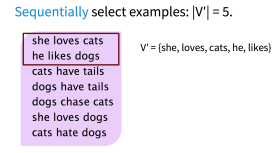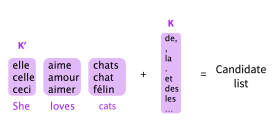### 6.3 Handling unknown words

1. learn to “copy” from source text (Pointer network, Gulcehre et al.)
1. Applies attention distribution $l_t$ to decide where to point in the source text and uses the decoder hidden state $S_t$ to predict a binary variable $Z_t$ which decides when to copy from source text.
2. The final prediction is either the word $y_t^w$ chosen by softmax over candidate list, or $y_t^l$ copied from source text depending on the value of $Z_t$.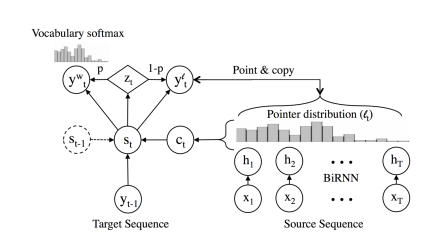this approach is both unreliable at scale - the attention mechanism is unstable when the network is deep - and copying may not always be the best strategy for rare words - sometimes transliteration is more appropriate.

## 7 Word and character-based models

Another direction to address unknown words is to operate at sub-word levels

• word segmentation
• character-based

Or, to embrace hybrid architectures

### 7.1 Word segmentation

• Representing rare and unknown words as a sequence of subword units.

• Byte Pair Encoding: start with a vocabulary of characters and keep extending the vocabulary with most frequent n-gram pairs in the data set.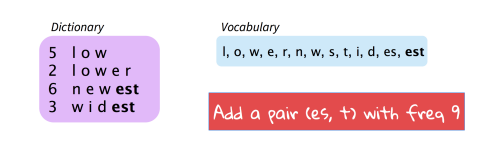• One can choose to either build separate vocabularies for training and test sets or build one vocabulary jointly.

### 7.2 Character-based model

• For each word $w$ with $m$ characters $c_1,c_2,\dots,c_m$ to look up the character embeddings $e_1, e_2, \dots e_m$ These character embeddings are then fed into a biLSTM to get the final hidden states $h_f, h_b$ . The final word embedding is computed by an affine transformation of two hidden states: $$e_w=W_fH_f + W_bH_b + b$$

### 7.3 Hybrid NMT

• Translates mostly at word-level and consults the character components for rare words.
• The character-level RNN compute source word representations and recover unknown target words when needed.
• Word-based Translation as a Backbone: word-level RNN use <UNK> for out of vocabulary words.
• Source Character-based Representation: character RNN process out of vocabulary word and feed final hidden state as embedding for the word for the word-level RNN.
• Target Character-level Generation. character RNN uses word-level model’s state(which leads to <unk>) as initial hidden and generates a word to replace <unk>

# Note 6 Self-Attention and Transformers

### RNN and Attention

• Attention in RNN: allow flexible access to memory (encoder hidden states)

• Issues with RNNs:

1. Linear interaction distance - takes O(seq length) for distant word pairs to interact.

• Hard to learn long-distance dependencies (gradient)

• Linear order of words is baked in, but we know linear order isn’t the right way to think about sentences. (Tree?)

2. Lack of parallelizability - forward and backward passes have O(seq length) unparallelizable operations.

• Need hidden states from previous step to compute current step
• Inhibits training on very large datasets

### Word Window and Attention

• Word windows:

• word window models aggregate local contexts (1D convolution)
• Number of un-parallelizable operations does not increase sequence length.
• Long-distance dependencies can be captured with stacking word window layers
• Maximum interaction distance = sequence length / window size
• Attention:

• Treats each word’s representation as a query to access and incorporate information from a set of values.
• Number of un-parallelizable opeartions does not increase sequence length.
• Maximum interaction distance: O(1), since all words interact at every layer.
• Self-Attention:

• queries, keys and values are drawn from the same source.

• simplest is to use v = k = q = x
• Dot product self-attention operation is as follows: \begin{align} e_{ij} = q_i^Tk_j & \qquad \text{compute key-query affinities} \\ \alpha_{ij} = \frac{exp(e_{ij})}{\sum_{j'}exp(e_{ij'})} & \qquad \text{compute attention weights from affinities (softmax)} \\ output_i = \sum_{j}\alpha_{ij}v_j & \qquad \text{compute output as weighted sum of values} \end{align}

• Can self-attention be a drop-in replacement for recurrence? No

1. self-attention is an operation on sets. It has no inherent notion of order.
• Add position embeddings to word embeddings. Used to be hand designed sinusoidal functions, now learned as well.
2. no nonlinearities for deep learning, it all just weighted averages.
• Add feed-forward network to post-process each output vector.
• Without it, stacking self-attention is just re-averages values.
3. Need to ensure we don’t “look at the future” when inference.
• Mask out attention to future words by setting attention scores to $-\infin$

### Transformer (Vaswani)

1. Key-Query-Value Attention: $$k_i = Kx_i, \qquad where \ K \in \mathbb{R}^{d\cross d}\\ q_i = Qx_i, \qquad where \ Q \in \mathbb{R}^{d\cross d}\\ v_i = Vx_i, \qquad where \ V \in \mathbb{R}^{d\cross d}\\$$ These matrices allow different aspects of the $x$ vectors to be used/emphasized in each of the three roles.

$X = [x_1;x_2;\dots;x_T] \in \mathbb{R}^{T\cross d}$ output is $softmax(XQ(XK)^T) \cross XV$

What if we want to look in multiple places in the sequence for different reasons all at once?

define multiple attention heads through multiple Q,K,V matrices.

Let $Q_l, K_l, V_l \in \mathbb{R}^{d\cross \frac{d}{h}}$ where $h$ is the number of attention heads.

Each attention head performs attention independently:

$output_l = softmax(XQ_lK_l^Tx^T)\cross XV_l$ where $output_l \in \mathbb{R}^{d/h}$

We then concatenate outputs to get back to the original dimension.

Each head gets to “look” at different things, and construct value vectors differently.

It is the same amount of compute as single-head attention.

3. Instead of $X^{i} = Layer(X^{i-1})$ let $X^{i} = X^{i-1} + Layer(X^{i-1})$

Residual connections are thought to make the loss landscape considerably smoother -> thus easier training.

4. Cut down on uninformative variation in hidden vector values by normalizing to unit mean and standard deviation within each layer

Maybe due to its normalizing gradients $$output = \frac{x-\mu}{\sqrt{\sigma} + \epsilon} * \gamma + \beta$$ where $\gamma$ and $\beta$ are learned “gain” and “bias”.

5. Scaled Dot Product Attention:

When $d$ becomes large, dot products tend to become large.

$output_l = softmax(\frac{XQ_lK_l^TX^T}{\sqrt{d/h}})\cross XV_l$

Query from Decoder and Key/Value from Encoder

• Drawbacks:
• Quadratic compute in self-attention. - due to all pair-wise interactions $O(T^2d)$
• Linformer map the sequence length dimension to a lower-dimensional space for values, keys
• BigBird

# Note 7 Subword, Transformer Pretraining

## Notes

### 1. Word Structure and subword models

• Traditional language model assumes a fixed vocab, built from the training set, all novel words seen at test time are mapped to a single UNK token.
• Finite vocabulary assumptions make even less sense in many languages, many languages exhibit complex morphology, or word structure. E.g a single verb may have hundreds of conjugations.
• Subword modeling:
• Dominant modern paradigm is to learn a vocabulary of parts of words (subword tokens).
• At training and testing time, each word is split into a sequence of known subwords.
• The byte-pair encoding algorithm
• Start with a vocabulary containing only characters and an “end-of-word” symbol.
• Using a corpus of text, find the most common adjacent characters “a,b”; add “ab” as a subword.
• Replace instances of the character pair with the new subword; repeat until desired vocab size.
• Common words splitted into subwords in vocab.
• Rare words splitted into subwords (sometimes intuitive, sometimes not), and in worst case, fallback to char stream.

### 2. Pretraining Word Embeddings

• Distributional semantics:

You shall know a word by the company it keeps - J. R. Firth

• But

the complete meaning of a word is always contextual, and no study of meaning apart from a complete context can be taken seriously - J. R. Firth

• Approach 1: Start with pretrained word embeddings only(no context, ie the same word will always get the same embedding no matter what context it is in.) and learn how to incorporate context in an LSTM or Transformer while training on the task.
• Issues:
• The downstream task may not be sufficient to teach all contextual aspects of language.
• Approach 2: All or almost all model parameters in the network are initialized via pretraining. Pretraining methods hide parts of the input from the model, and train the model to reconstruct those parts.
• This filling the blank pretraining is effective at building strong:
• representations of language
• parameter initializations for strong NLP models
• Probability distributions over language that we can sample
• Language modeling as pretraining:
•  Model $p_{\theta}(w_t w_{1:t-1})$ the probability distribution over words given their past contexts.
• Pretraining model (optionally and word embedding) on language modeling task, then fine tune on the task at hand (maybe sentiment classifier).
• Stochastic gradient descent and pretrain/finetune
• Pretraining matter because SGD sticks close to the parameters $\hat{\theta}$ from pretraining during fine tuning(if lr is small of course).
• Either:
• Fine tuning near the local minima near $\hat{\theta}$ tend to generalize well.
• And/Or, maybe the gradients of finetuning loss near $\hat{\theta}$ propagate nicely.
• Three pretraining schemes:
• Decoders only - Language models, nice to generate from, but can’t condition on future words
• Encoders only - Gets bidirectional context - can condition on future
• Encoder-Decoders - Good parts from above? but how do we train this

### 3. Decoder only pretraining

• Train decoder only language model then tune on classification / QA / summarization task.
• GPT(Radford et al. 2018) is decoder only transformer pretrained as language models.
• 12 Layers of transformer decoders
• 768 - dimensional hidden states, 3072-dimensional feed-forward hidden layers
• Byte-pair encoding with 40,000 merges
• Trained on BooksCorpus: over 7000 unique books
• GPT = Generative PreTraining / Generative Pretrained Transformer
• Natural Language Inference - label pairs of sentences as entailing / contradictory / neutral
• [START] The man is in the doorway [DELIM] The person is near the door [EXTRACT]
• The linear classifier is applied to the representation of the [EXTRACT] token.
• The next generation GPT2 showed better natural language generations.

### 4. Encoder only pretraining

• Since encoders get bidirectional context, we can’t do language modeling. Instead we predict masked words. Called Masked LM.

•  Only add loss terms from words that are “masked out”. Learn $p_{\theta}(x \tilde{x})$ where $\tilde{x}$ is the masked version of the sequence.
• BERT (Devlin et al, 2018) is encoder only transformer pretrained on masked language model objective.
• Predict a random 15% of (sub)word tokens.
• Replace input word with [MASK] 80% of the time
• Replace input word with a random token 10% of the time
• Leave input word unchanged 10% of the time (but still predict it)
• Why? Doesn’t let the model get complacent and not build strong representations of non-masked words.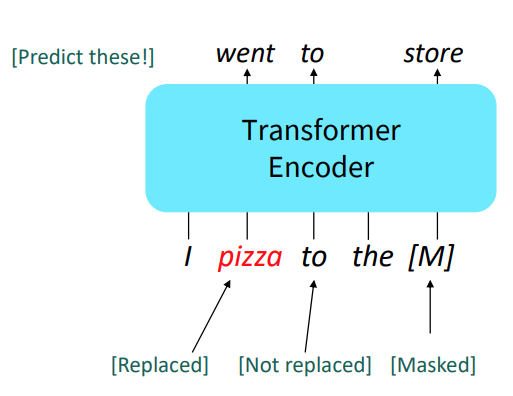• The pretraining input to BERT was two separate contiguous chunks of text:
[CLS]   my      dog     is      cute    [SEP]   he      likes   play    ##ing   [SEP]
A		A		A		A		A		A		B		B		B		B		B
0       1       2       3       4       5       6       7       8       9       10

• Two released model:
• base - 12 layers, 768-dim hidden states, 12 attention heads, 110 million params
• large - 24 layers, 1024-dim hidden states, 16 attention heads, 340 million params
• Trained on Books Corpus + English wikipedia
• Trained on 64 TPU chips
• Fine tuning is practical and common on a single GPU
• Limitations of pretrained encoders

• pretrained encoders don’ naturally lead to nice autoregressive (1-word-at-a-time) generation methods.
• BERT extensions: like RoBERTa, SpanBERT, etc

• RoBERTa: train BERT for longer and remove next sentence prediction! More compute, more data can improve pretraining even when not changing the underlying transformer encoder.
• SpanBERT: masking contiguous spans of words makes a harder, more useful pretraining task

### 5. Encoder-Decoder pretraining

• Encoder portion can benefits from bidirectional context; and the decoder portion is used to train the whole model through language modeling.
• Objective: Span corruption(denoising). T5 (Raffel et al, 2018)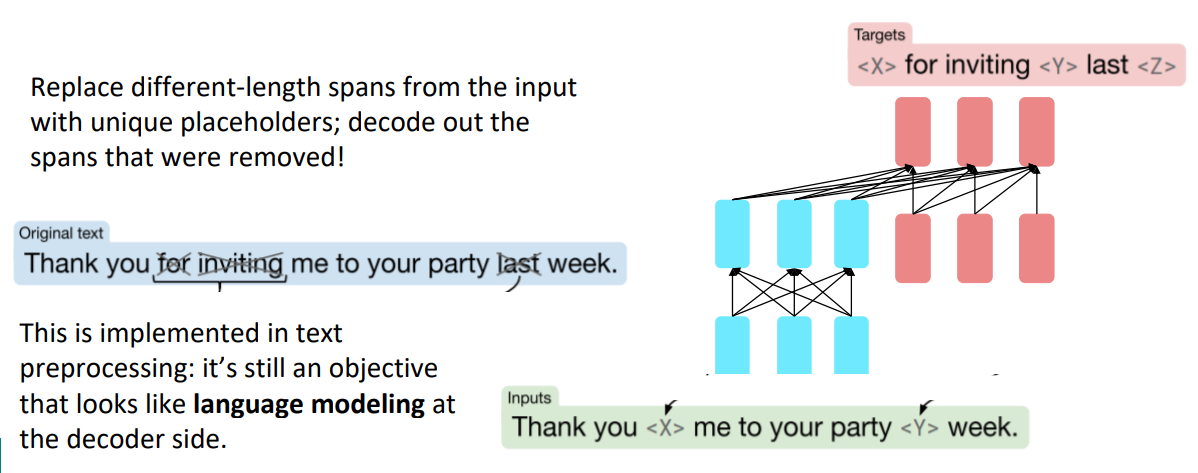• They found encoder-decoder work better than decoders only, and span corruption work better than language modeling.
• T5 was tuned on wide range of QA tasks, NQ(Natural Questions), WQ(WebQuestions), TQA(Trivia QA).

### 6. What do we think pretraining is teaching?

• There is increasing evidence that pretrained models learn a wide variety of things about the statistical properties of language.
• Stanford University is located in __, California. [Trivia]
• I put _ fork down on the table. [syntax]
• The woman walked across the street, checking for traffic over _ shoulder. [coreference]
• I went to the ocean to see the fish, turtles, seals, and _____. [lexical semantics/topic]
• Overall, the value I got from the two hours watching it was the sum total of the popcorn and the drink. The movie was _. [sentiment]
• Iroh went into the kitchen to make some tea. Standing next to Iroh, Zuko pondered his destiny. Zuko left the . [some reasoning – this is harder]
• I was thinking about the sequence that goes 1, 1, 2, 3, 5, 8, 13, 21, [some basic arithmetic; they don’t learn the Fibonnaci sequence]
• Models also learn – and can exacerbate racism, sexism, all manner of bad biases.

### 7. Very Large Models

• Two ways of interact with pretrained models:
• Sample from the distributions they define(maybe providing a prompt)
• Fine-tune them on a task we care about, and take their predictions
• Very large language models seem to perform some kind o f learning without gradient steps simply from examples you provide within their contexts.
• GPT-3 is the canonical example of this, 175 billion parameters. (T5 is 11 billion)
• The in-context examples seem to specify the task to be performed, and the conditional distribution mocks performing the task to a certain extent.
• input: “thanks->merci; hello->bonjour; mint->menthe; otter -> “ output “loutre”

## Notes

### Overview

• build systems that automatically answer questions posed by humans in a natural language
• Question types
• Factoid vs non-factoid, open-domain vs closed domain, simple vs compositional
• short segment of text, a paragraph, a list, yes/no, …
• IBM Watson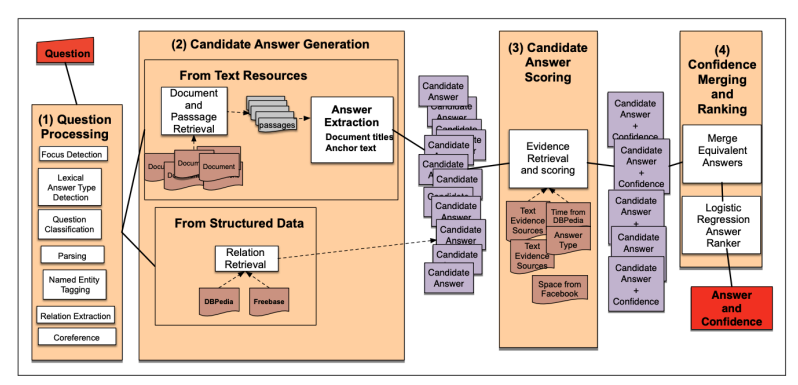• Deep learning era: all SOTA QA systems are built on top of end-to-end training and pre-trained language models.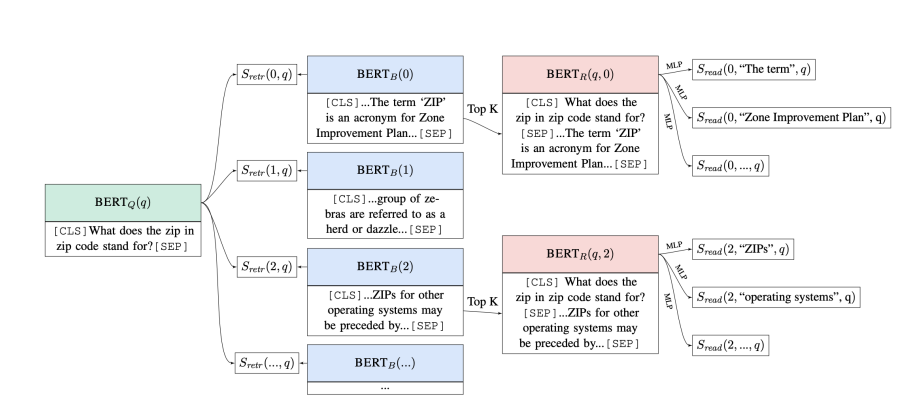• There are also QA system built with knowledge graphs.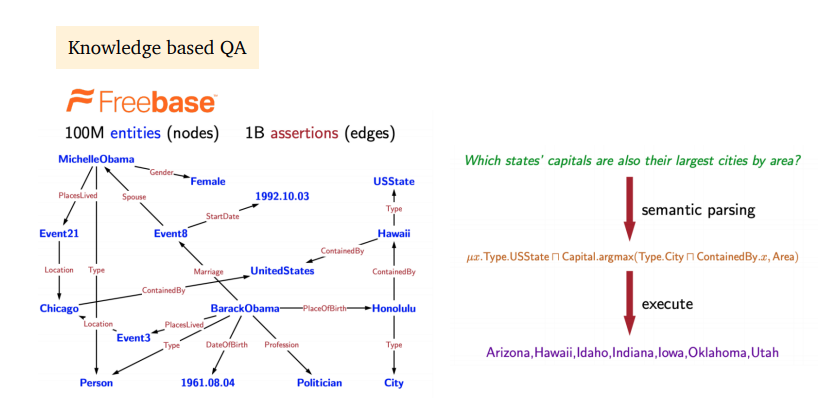• comprehend a passage of text and answer questions about its content $(P, Q) \rightarrow A$
• many other NLP tasks can be reduced to a reading comprehension problem:
• Information extraction:
• Barack Obama, educated_at, ? -> where did Barack Obama graduate from?
• Semantic role labeling
• etc
• 100k annotated (Passage, Question, Answer) triples
• Passages are from English Wikipedia, usually 100~150 words
• Questions are crowd-sourced
• Each answer is a span in the passage - not all questions can be answered in this way. 3 gold answers per questions
• Evaluation is exact match(0/1) or F1
• Human reference: EM 82.3% F1 91.2
• Problem formulation:
• Input: $C=(c_1, c_2, \dots, c_N), Q=(q_1, q_2, \dots, q_n)$
• Output: $1 \leq start \leq end \leq N$
• 2016-2018: A family of LSTM-based models with attention
• 2019+: Fine-tuning BERT-like models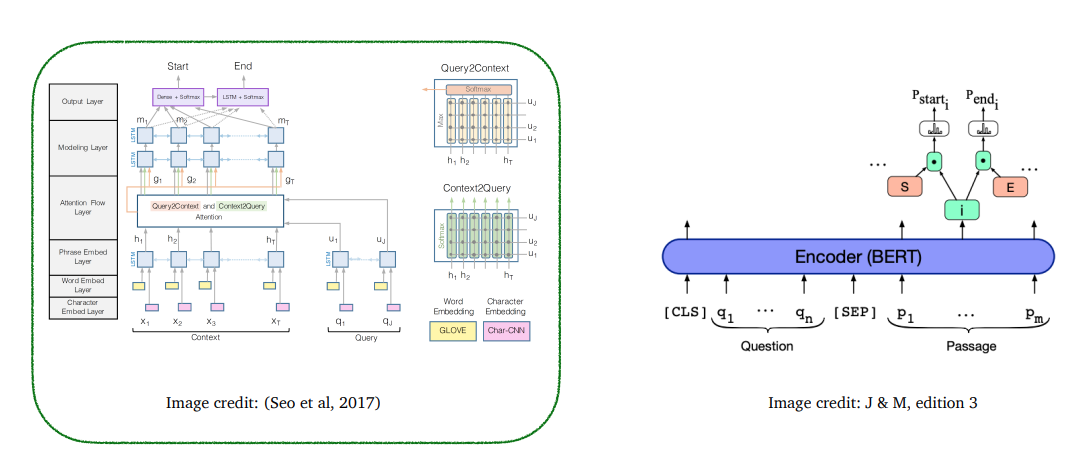### BiDAF: the Bidirectional Attention Flow model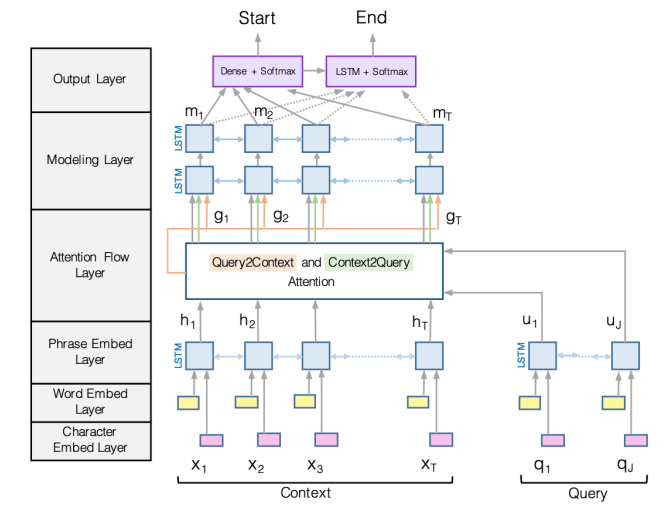• Encoding:

• Use a concatenation of word embedding (GloVe) and character embedding (CNNs over character embeddings) for each word in context and query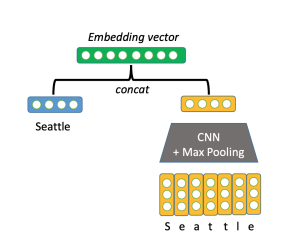$e(c_i) = f([GloVe(c_i);charEmb(c_i)])$

• Then use two bidirectional LSTMs separately to produce contextual embeddings for both context and query

$\overrightarrow{c_i} = LSTM(\overrightarrow{c_{i-1}}, e(c_i)) \in \mathbb{R}^H \\ \overleftarrow{c_i} = LSTM(\overleftarrow{c_{i+1}}, e(c_i)) \in \mathbb{R}^H \\ c_i = [\overrightarrow{c_i}; \overleftarrow{c_i}] \in \mathbb{R}^{2H}$
• Attention:

• Context-to-query attention: for each context word, choose the most relevant words from the query words

• Query-to-context attention: choose the context words that are most relevant to one of the query words

• Compute:

• First, similarity score for every pair of $(c_i, q_j)$: $$S_{i,j} = w^T_{sim}[c_i;q_j;c_i\odot q_j] \in \mathbb{R} \qquad w_{sim} \in \mathbb{R}^{6H}$$

• Context-to-query attention (which question words are more relevant to $c_i$): $$\alpha_{i,j} = softmax_j(S_{i,j}) \in \mathbb{R} \qquad a_i = \sum_{j=1}^{M}\alpha_{i,j}q_j\in \mathbb{R}^{2H}$$

• Query-to-context attention (which context words are relevant to some question words): $$\beta_{i} = softmax_i(max_{j=1}^{M}(S_{ij})) \in \mathbb{R}^N \qquad b=\sum_{i=1}^{N}\beta_{i}c_{i} \in \mathbb{R}^{2H}$$

• The final output is: $$g_i = [c_i;a_i;c_i\odot a_i;c_i\odot b] \in \mathbb{R}^{8H}$$

• Modeling Layer:

• pass $g_i$ to another two layers of bi-directional LSTMs

• Attention layer is modeling interactions between query and context

• Modeling layer is modeling interactions within context words(annotated with query?)

$m_i = BiLSTM(g_i)\in \mathbb{R}^{2H}$

• Output Layer:

• two classifier

• s predicting the start and end position: $$p_{start} = softmax(w^T_{start}[g_i;m_i]) \\ p_{end} = softmax(w^T_{end}[g_i;m'_i]) \\ m'_i = BiLSTM(m_i) \in \mathbb{R}^{2H} \\ w_{start}, w_{end} \in \mathbb{R}^{10H}$$

• Performance:

• 77.3 F1 on SQuAD v1.1
• 67.7 F1 without context-to-query attention
• 73.7 F1 without query-to-context attention
• 75.4 F1 without character embeddings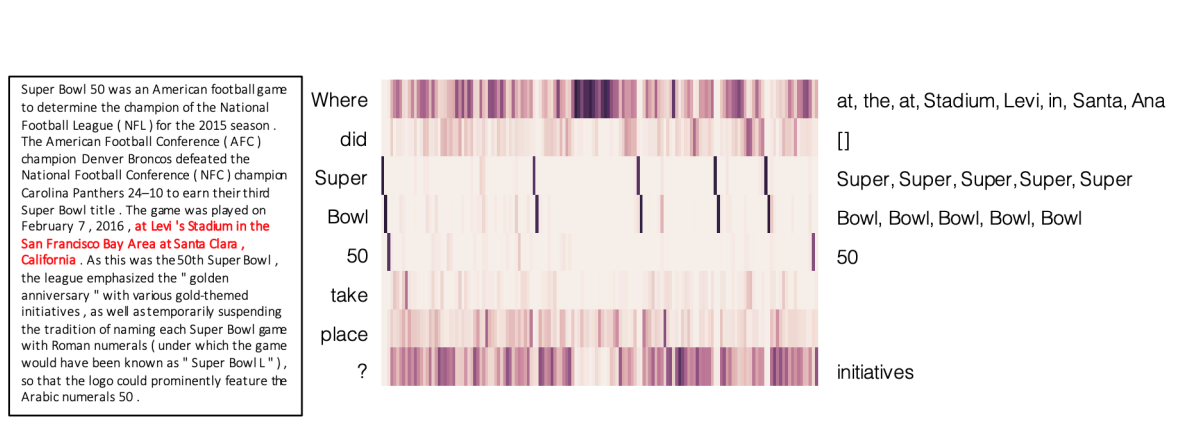• Question = Segment A
• Passage = Segment B
• Answer = predicting two endpoints in segment B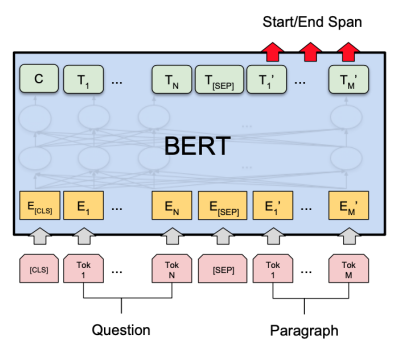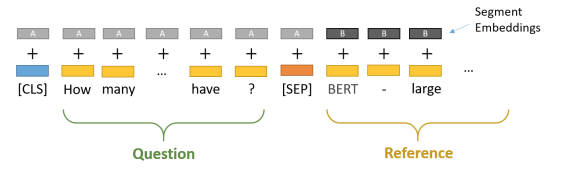$$L = -logp_{start}(S^*) - logp_{end}(e^*) \\ p_{start}(i) = softmax_i(w^T_{start}H) \\ p_{end}(i) = softmax_i(w^T_{end}H) \\ where \ H = [h1,h2,\dots,h_N] \\ \text{are hidden vectors of the paragraph, returned by BERT}$$

• Performance: beat human reference

### Compare BiDAF and BERT

• BERT is 100 times larger than BiDAF in terms of #parameters
• BiDAF is attention connected multiple LSTM models, whereas BERT is Transformer.
• But, fundamentally they are not much different:
• BiDAF models attention of (P,Q) and (Q, P)
• BERT models attention of (P,Q), (Q, P) and (Q, Q) and (P, P) on top of BiDAF, through its self attention.
• Can we design better pre-training objectives for BERT for QA? - SpanBERT (Joshi & Chen et al, 2020)
• using two end points of span to predict all the masked words in between = compressing the information of a span into its two endpoints.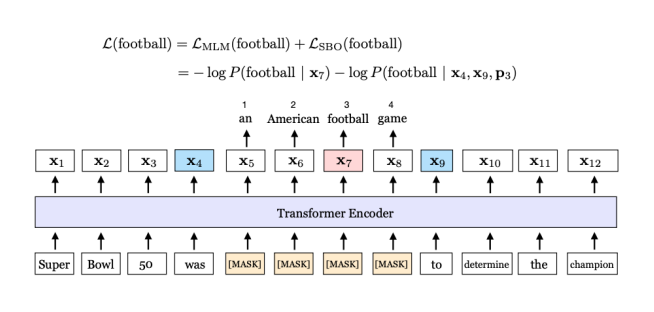### Is reading comprehension solved? No

• Even though BERT already out-performed human on SQuAD, but the systems still perform pooly on adversarial examples or examples from out-of domain distributions.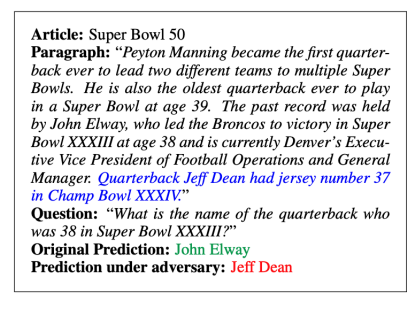• Also, systems trained on one dataset can’t generalize to other datasets.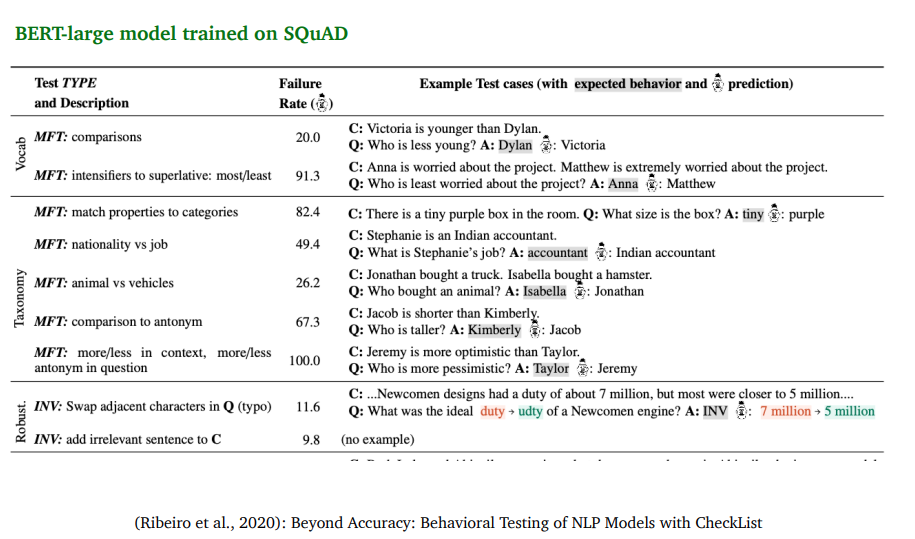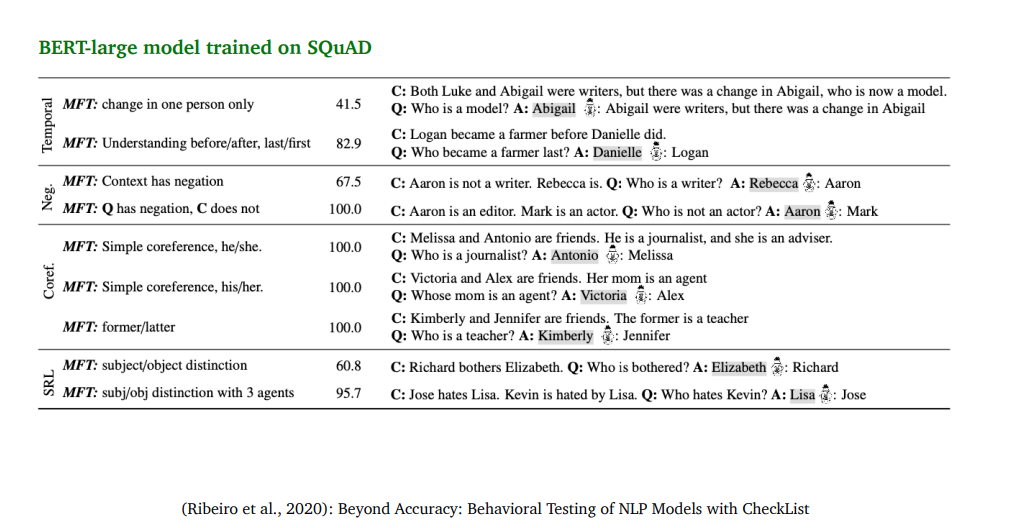• Different from reading comprehension, we don’t assume a given passage

• Only have access to a large collection of documents, we don’t know where the answer is located.

• Input: a large collection of documents $\mathit{D}=D_1, D_2, \dots, D_N$ and $Q$
• Output: an answer string $A$
• Retriever: $f(D, Q) \rightarrow P_1, \dots, P_K$ K is pre-defined (e.g. 100)
• Reader: $g(Q, {P_1, \dots, P_K}) \rightarrow A$ A is a reading comprehension problem
• In Dr.QA:

• Retriever = A standard TF-IDF information-retrieval sparse model ( a fixed module)
• Latent Retrieval for Weakly Supervised Open Domain Question Answering (Lee, et al, 2019)• Joint training of retriever and reader
• Each text passage can be encoded as a vector using BERT and the retriever score can be measured as the dot product between the question representation and passage representation
• However, it is not easy to model as there are huge number of passages
• Dense Passage Retrieval for Open-Domain Question Answering (Karpukhin et al. 2020)

• DPR - train the retriever using question-answer pairs
• Trainable retriever largely outperforms traditional IR retrieval models. (1K pairs beats BM25)
• Leveraging Passage Retrieval with Generative Models for Open Domain Question Answering

• Fusion-in-decoder (FID) = DPR + T5
• How Much Knowledge Can You Pack Into the Parameters of a Language Model? (Roberts et al, 2020)

• Large Language models can do open-domain QA well
• without an explicit retriever stage
• Tuning T5
• Real-Time Open-Domain Question Answering with Dense-Sparse Phrase Index (Seo et al, 2019)

• Learning Dense Representations of Phrases at Scale (Lee, et al, 2020)

• It is possible to encode all the phrases using dense vectors and only do nearest neighbor search without a BERT model at inference time

# Note 9 Natural Language Generation

## Notes

• Examples of NLG:

• Machine Translation
• Dialogue Systems
• Summarization
• Data-to-Text Generation
• Visual Description
• Creative Generation
• NLG: any task involving text production for human consumption.

• Basics of NLG

• Autoregressive model: predict $\hat{y}t = f({y{<t}})$
•  Score $S \in \mathbb{R}^{ V } = f({y_{ • Distribution$P$over$w \in V$:$P(y_t=w|{y_{<t}})=\frac{exp(S_w)}{\sum_{w’\in W}exp(S_{w’ })}$•  Training: minimizing$L_t = -logP(y_t {y_{
•  Inference: $\hat{y}_t=g(P(y_t {y_{ • Decoding algorithms • Greedy methods: •  argmax decoding:$\hat{y}_t = \underset{w\in W}{argmax}P(y_t=w y_{y
• Beam Search:

• Keep a
• Dealing with repetition:

• Heuristic: Don’t repeat n-grams
• Complex:
• Minimize embedding distance between consecutive sentences: does not help with intra-sentence repetition
• Coverage loss: prevents attention from attending to the same words
• Unlikelihood objective: Penalize generation of already-seen tokens
• Greedy methods heavily focus on high probable sequence, not random enough to match human.

• Top-k sampling:

• Sample from the top k tokens in the probability distribution
• Increase k for more diverse / risky outputs
• Decrease k for more generic / safe outputs
• Problem:
• The distributions we are sample from are dynamic
• When P is flat, limited k removes many viable options
• When P is peakier, a high k allows for too many options
• Top-p (nucleus) sampling:

• Sample from all tokens in the top p cumulative probability mass
• Varies k depending on the uniformity of P
• Scaling randomness: Softmax temperature $$P_t(y_t=w) = \frac{exp(S_w/\tau)}{\sum_{w' \in V}exp(S_{w'}/\tau)}$$ Raise the temperature $\tau > 1$: $P$ becomes more uniform -> more diverse output

Lower the temperature $\tau < 1$: $P$ becomes more spiky -> less diverse output

temperature is hyper-parameter for decoding methods

• Re-balancing distributions

• Re-balance $P$ using retrieval from n-gram phrase statistics
• Back propagation-based distribution re-balancing:
• Latent –foward–> sentiment scoring model
• Latent <-backward- loss
• Latent –decoder–> P
• Re-ranking
• Generate a bunch sequences, use one or more re-rankers to score / rank them and pick one
• Different decoding algorithms can allow us to inject biases that encourage different properties of the generated text.

# Note 10 Coreference Resolution

## Notes

### What is Coreference Resolution?

• Identify all mentions that refer to the same entity in the word

• Step 1: Detect the mentions
• Step 2: Cluster the mentions
• Mention Detection: A span of text referring to some entity

• Pronouns
• Named entities
• Noun phrases
• Keep all mentions as candidates, running them through coreference system and discard singleton mentions.

• Or we can train end-to-end model

• Four kinds of coreference models:

• Rule-based (pronominal anaphora resolution)
• Hobbs’ naive algorithm 1976
• Mention Pair (binary classification)
• Mention Ranking (softmax)
• Clustering
• Non-Neural Coref Model: Features

• Person / Number / Gender agreement
• Semantic compatibility
• Certain syntactic constraints
• More recently mentioned entities preferred for referenced
• Grammatical Role: Prefer entities in the subject position
• Parallelism
• Neural Coref Model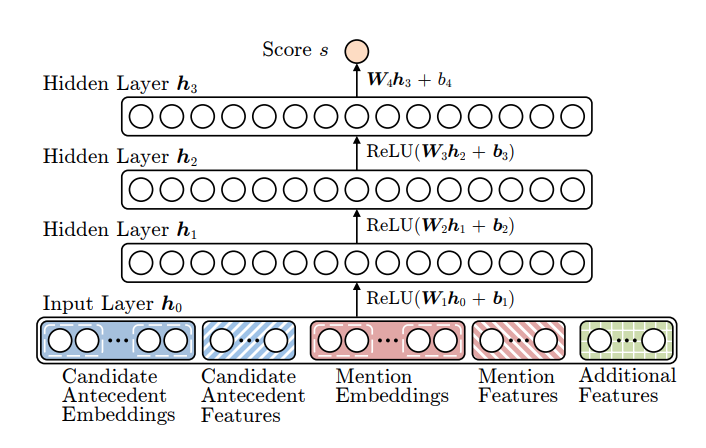• Embeddings:
• previous two words, first word, last word, head word etc of each mention
• distance, document genre, speaker information etc

### Convolutional Neural Nets

• 1d discrete convolution definition:

$(f*g)[n] = \sum_{m=-M}^Mf[n-m]g[m]$

• Convolution is classically used to extract features from images
• batch_size = 16
word_embed_size = 4
seq_len = 7
x = torch.randn(batch_size, word_embed_size, seq_len)
conv1 = Conv1d(in_channels=word_embed_size, out_channels=3, kernel_size=3, padding=1)
hidden1 = conv1(x)
hidden2 = torch.max(hidden1, dim=2) # max pooling

• Models position-invariant identification
• filter is of size: kernel_size x in_channels
• number of filters is the same as number of desired output channels
• output seq len depends on padding, kernel size and seq_len as well as stride

### End-to-end Model

• Embed words in the document using a word embedding matrix and a character-level CNN
• Then run a bidirectional LSTM over the document
• Next, represent each span of text i going from start(i) to end(i) as a vector
• Span representation: $g_i=[x^_{start(i)},x^_{end(i)},\hat{x_i},\phi(i)]$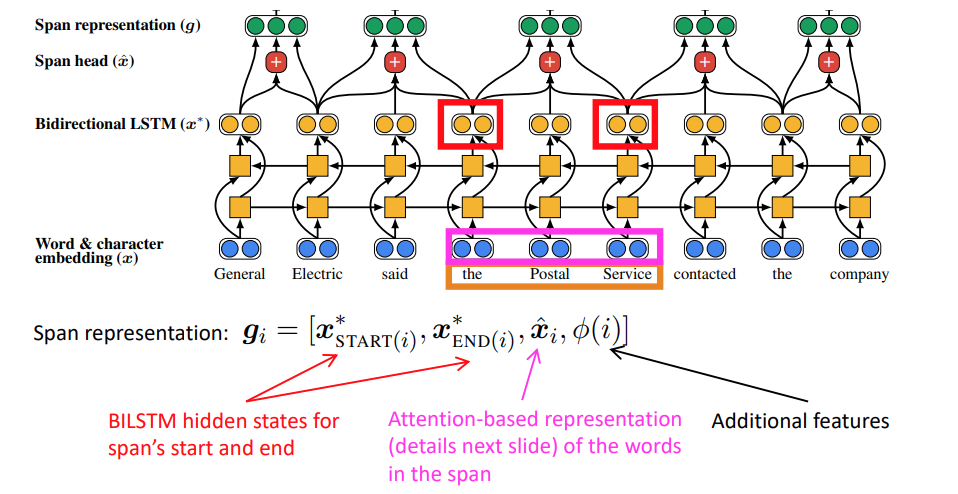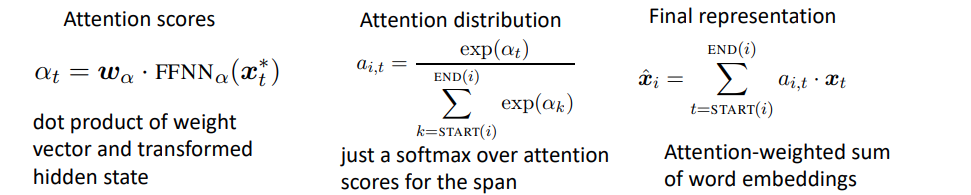• $x^*_{start(i)}$ gives the context to the left
• $x^*_{end(i)}$ gives the context to the right
• $\hat{x_i}$ gives the representation of the span
• $\phi(i)$ gives other information in the text

• Lastly score every pair of spans: $$s(i, j) = s_m(i) + s_m(j) + s_a(i,j) \\ s_m(i) = w_m \cdot FFNN_m(g_i) \\ s_a(i, j) = w_a \cdot FFNN_a([g_i, g_j, g_i \odot g_j, \phi(i, j)])$$

• Intractable to score every pair of spans

• $O(T^2)$ spans of text in a document
• $O(T^4)$ runtime
• Lots of pruning to work on
• Attention learns which words are important in a mention (like head words)

### BERT-based coref:

• SpanBERT: pretrains BERT models to be better at span-based prediction tasks like coref and QA
• BERT-QA for coref: Treat Coreference like a deep QA task:
• Point to a mention, and ask “what is its antecedent”?

### Coreference Evaluation

• Many different metrics: MUC, CEAF, LEA, B-CUBED, BLANC, F1 score average over 3 coreference metrics
• Dataset: OntoNotes dataset ~ 3000 documents labeled by humans

# Note 11 T5 and Large Language Models

## Notes

### Which transfer learning methods work best and what happens when we scale them up?

• T5 - Text-to-Text Transfer Transformer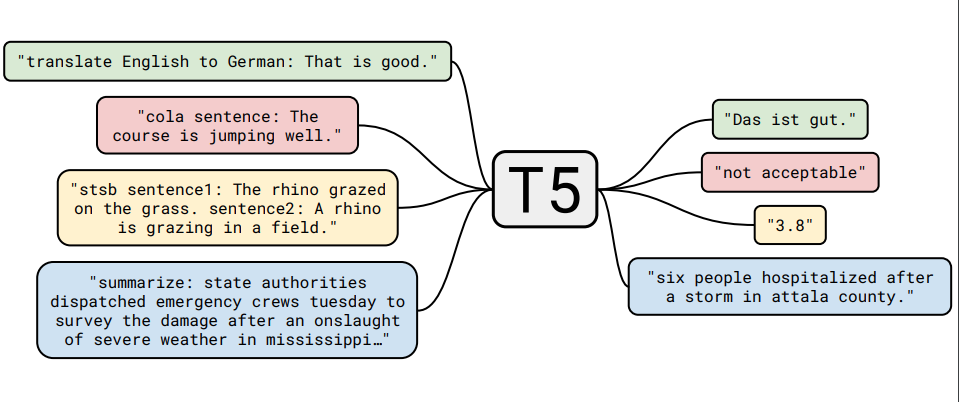• Architecture: standard BERT

• Dataset: C4 (processed common crawl)

• Pretraining task: Span prediction (Denoising objective)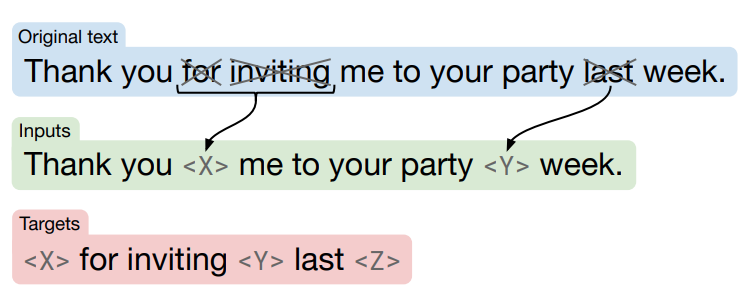• Multitask learning, how often I sample from each task? Often hurt single task performance compare to tune single task. Each task has its own prefix(like “stsb”, “summarize” etc)
• Best-strategy: Multitask learning with task data + unsupervised pretraining data -> fine tuning on single tasks
• Scale:
• larger model train longer > even larger model > training even longer > ensemble!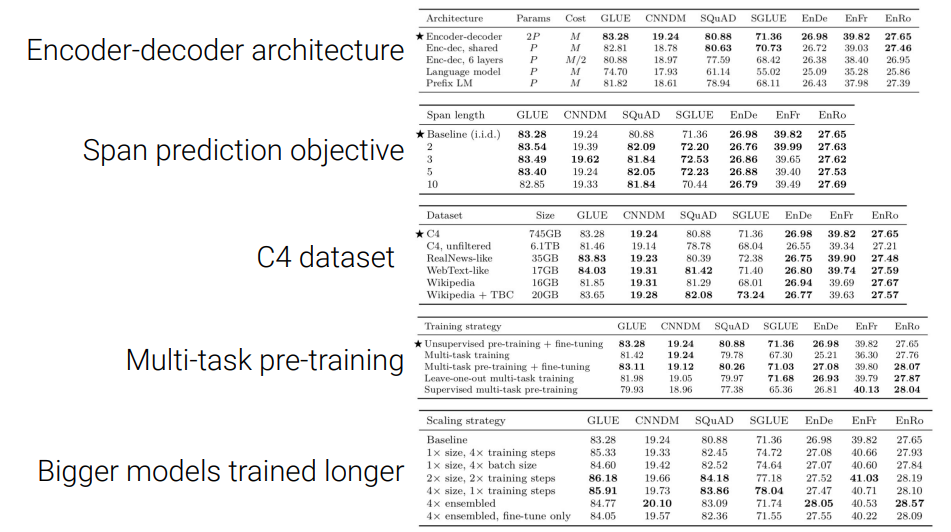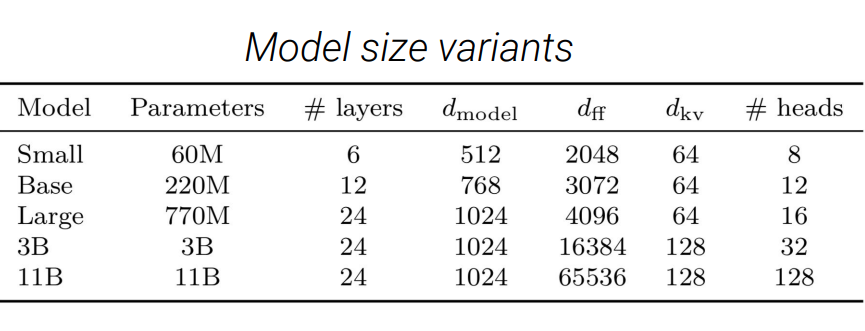### How much knowledge does a language model pick up during pre-training?

• pretrained T5 LM tested on closed-book QA(no retrieval)

• Gap behind SOTA systems, but larger model score better -> suggests larger model pick up more knowledge?

• Add Salient Span Masking - REALM: Retrieval-Augmented Language Model Pre-Training (Guu et al)

Greatly improved QA close to SOTA

• How do we know when language model know? - highly calibrated model will not produce high confident score for the predictions when it does not know the answer.

• Fun fact: T5 peaked downstream task performance before a single pass on the tuning set. -> unlikely the model overfits the down stream task data.

### Do large language models memorize their training data?

• GPT2 has memorized its training data. Prefix East Stroudsburg Stroudsburg->

• Larger model memorize more data, even when the data only occur very rarely…

### Can we close the gap between large and small models by improving the Transformer archtecture?

• Modifications:
1. Factorized embedding
2. Shared embedding and softmax layer
3. Mixture of Softmaxes / adaptive Softmax
4. Different way of initializing / normalizing the model
5. Different attention mechanism
6. Different structure for the feed forward network: mixture of experts, switch transformer, different nonlinearities.
7. Funnel Transformer, Evolved Transformer, Universal Transformer, block sharing.
• Nothing work obviously better. Nothing statistically significant

### Reference

1. https://arxiv.org/abs/1910.10683
2. https://arxiv.org/abs/2010.11934
3. https://arxiv.org/abs/2002.08910
4. https://arxiv.org/abs/2012.07805
5. https://arxiv.org/abs/2102.11972

# Note 12 Integrating Knowledge in Language Model

## Notes

### Recap Language Models

• Standard is to predict next word; recently the masked language models predict masked tokens
• Used to do language generation or evaluating the probability of text:
• summarization
• dialogue
• autocompletion
• machine translation
• fluency evaluation
• Can a language model be used as a knowledge base? (Petroni et al. 2019)
• predictions generally make sense (e.g. the correct types), but are not all factually correct
• maybe due to:
• unseen facts: fact not in training corpora
• rare facts: hasn’t seen enough examples to memorize the fact
• model sensitivity: e.g. can answer ‘x was made in y’, but not ‘x was created by y’
• Unable to reliably recall knowledge
• Knowledge-aware language models
• LM can benefit downstream tasks that leverage knowledge
• e.g. entity relation extraction
• Can language model replace knowledge base?
• Pro:
• LM trained on unlabeled data; KB is manual annotated or complex NLP pipelines
• LM support more flexible natural language queries; KB query by triplets
• Cons:
• LM is hard to interpret, why it says so?
• LM is hard to modify, thinking about retrain to fix a single error?

### Techniques to add knowledge to LMs

• Categories

• ERNIE
• KnowBERT
2. Use a external memory
• KGLM
• KNN-LM
3. Modify the training data
• WKLM
• ERNIE, - salient span masking
• 1 Add pretrained entitiy embeddings

• USA, US, United States will have different word embeddings, but should have same entity embedding. If we can correctly link the word token of entity to its entity id. We can incorporate pretrained entitiy embeddings trained via:

• Knowledge graph embedding methods - TransE
• Word-entity co-occurrence methods - Wikipedia2Vec
• Transformer encodings of entity descriptions - BLINK
• How do we incorporate pretrained entitiy embeddings from a different embedding space?

• Learn a fusion layer to combine context and entity information

$h_j = F(W_tw_j + W_ee_k + b)$ where $w_j$ is the word embedding and $e_k$ is the entity embedding

• ERNIE: Enhanced Language Representation with Informative Entities (Zhang et al. ACL 2019)

• Text encoder: BERT like

• Knowledge encoder: stacked blocks composed of:

• Two multi-headed attentions (MHAs) over entity embeddings and token embeddings

• A fusion layer to combine the output of the MHAs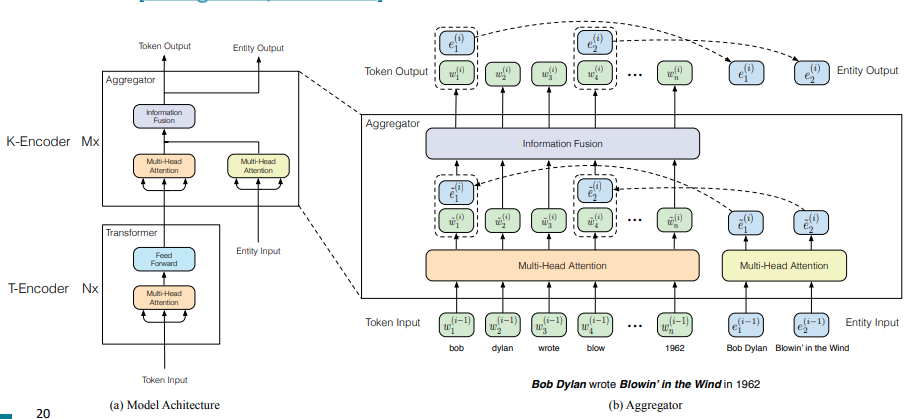• Trained with BERT objective + a knowledge pretraining task (dEA - denoising entity autoencoder, Vincent et al. ICML 2008): randomly mask token-entity alignments and predict the corresponding entity for a token from the entities in the sequence.

• Remaining challenges:

• Needs text data with entities annotated in the input.
• KnowBERT: Jointly learn to link entities with KnowBERT (Peters et al. EMNLP 2019)

• pretrain an integrated entity linker (EL) as an extension to BERT
• EL predicts entities so entity annotations aren’t required
• Learning EL may better encode knowledge
• Also uses a fusion layer to combine entity and context information and adds a knowledge pretraining task.
• 2 Use an external memory

• Are their more direct ways than pretrained entity embeddings to provide the model factual knowledge?

• Can better support injecting and updating factual knowledge
• More interpretable
• Knowledge-Graphs for Fac-Aware Language Modeling (Logan et al. ACL 2019)

• Key idea: condition the language model on a knowledge graph
 $P(x^{t+1}, \epsilon^{t+1} x^{t-m}, \epsilon^{t-m},\dots,x^{t}, \epsilon^{t})$ where $\epsilon^{t}$ is the KG entities mentioned at timestep $t$
• Build a “local” knowledge graph as you iterate over the sequence
• Local KG: subset of the full KG with only entities relevant to the sequence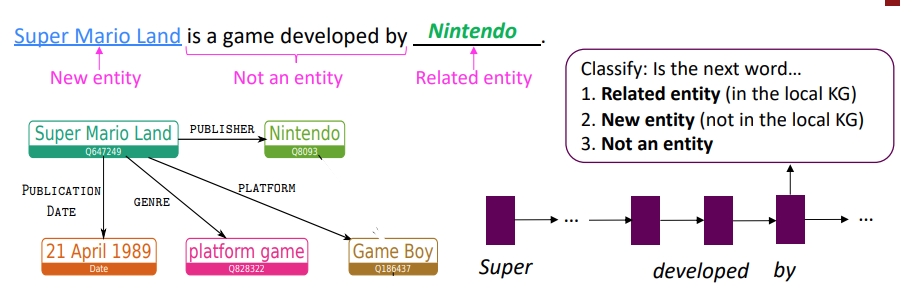• LSTM hidden state used to predict the types of the next word:
• Related Entity (in the local KG)
• Find the top-scoring parent and relation in the local KG using the LSTM hidden state and pretrained entity and relation embeddings
• $P(p_t) = softmax(v_p \cdot h_t)$, where $p_t$ is the “parent” entity, $v_p$ is the corresponding entity embedding, and $h_t$ is from the LSTM hidden state
• Next entity: tail entity from KG triple of (top parent entity, top relation, tail entity)
• Next word: most likely next token over vocabulary expanded to include entity aliases.
• New entity ( not in the local KG)
• Find the top-scoring entity in the full KG using the LSTM hidden state and pretrained entity embeddings
• Next entity: directly predict top-scoring entity
• Next word: most likely next token over vocabulary expanded to include entity aliases
• Not an entity
• Next entity: None
• Next word: most likely next token over standard vocabulary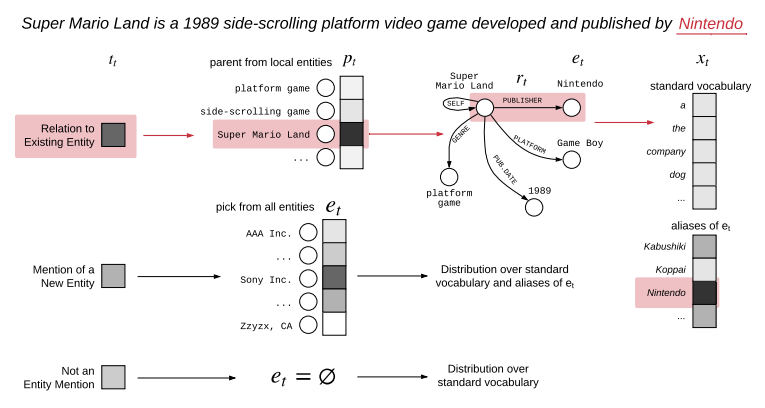• KGLM outperforms GPT-2 and AWD-LSTM on a fact completion task

• Qualitatively, compared to GPT-2, KGLM tends to predict more specific tokens

• Nearest Neighbor Language Models (KNN-LM) (Khandelwal et al ICLR 2020)

• Key idea: learning similarities between text sequences is easier than predicting the next word

• Example: “Dickens is the author of _” $\approx$ “Dickens wrote _”

• Qualitatively, researchers find this especially true for “long-tail patterns”, such as rare facts

• So, store all representations of text sequences in a nearest neighbor datastore

• At inference:

• Find the k most similar sequences of text in the datastore

• Retrieve the corresponding values (i.e. the next word) for the k sequences

• Combine the KNN probabilities and LM probabilities for the final prediction

 $P(y x) = \lambda P_{KNN}(y x) + (1-\lambda)P_{LM}(y x)$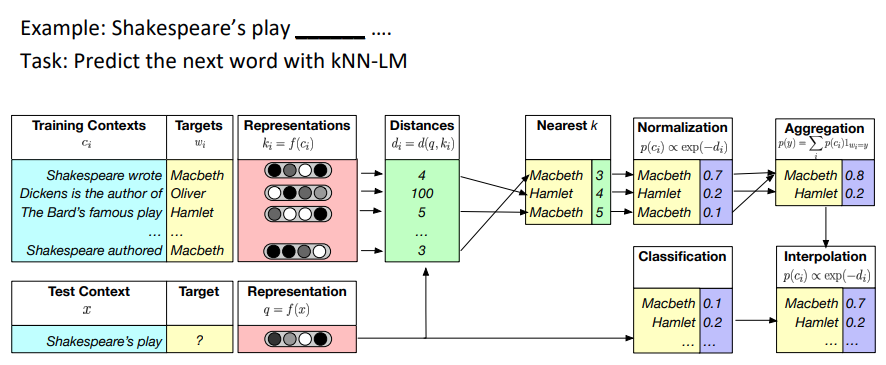• 3 Modify the training data

• Question: Can knowledge also be incorporated implicitiy through the unstructured text?

• No additional memory / computation requirements
• No modification of the architecture required
• Pretrained Encyclopedia: Weakly Supervised Knowledge Pretrained Language Model(WKLM) (Xiong et al. ICLR 2020)

• Key idea: train the model to distinguish between true and false knowledge
• Replace mentions in the text with mentions that refer to different entities of the same type to create negative knowledge statements (Swap noise???)
• Model predicts if entity as been replaced or not
• Type-constraint is intended to enforce linguistically correct sentences
• eg.
• True knowledge statement: J.K. Rowling is the author of Harry Potter
• Negative knowledge statement: J.R.R Tolkien is the author of Harry Potter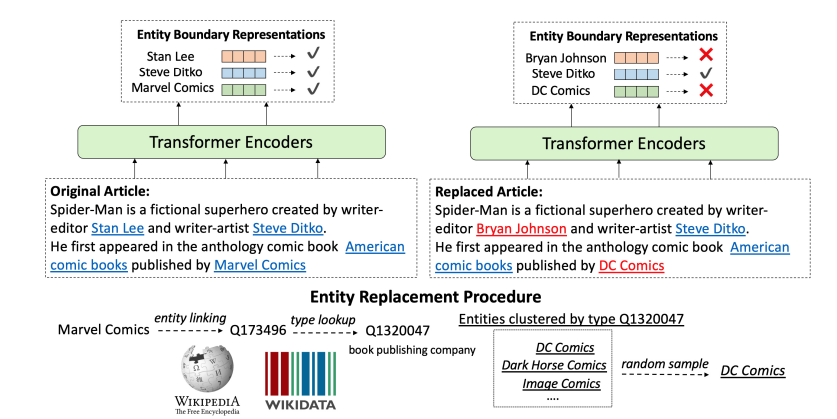• Uses an entity replacement loss to train the model to distinguish between true and false mentions

 $L_{entRep} = \prod_{e\in\epsilon^{+}}logP(e C) + (1- \prod_{e\in\epsilon^{+}})log(1-P(e C))$
• Total loss is the combination of standard masked language model loss and the entity replacement loss

$L_{WKLM} = L_{MLM} + L_{entRep}$

• MLM is defined at the token level, entRep is defined at the entity level.

• ERNIE: Enhanced Representation through Knowledge Integration

• Learn inductive biases through masking
• Can we encourage the LM to learn factual knowledge by being clever about masking?
• phrase level and entity level masking
• salient span masking to mask out salient spans (named entities and dates)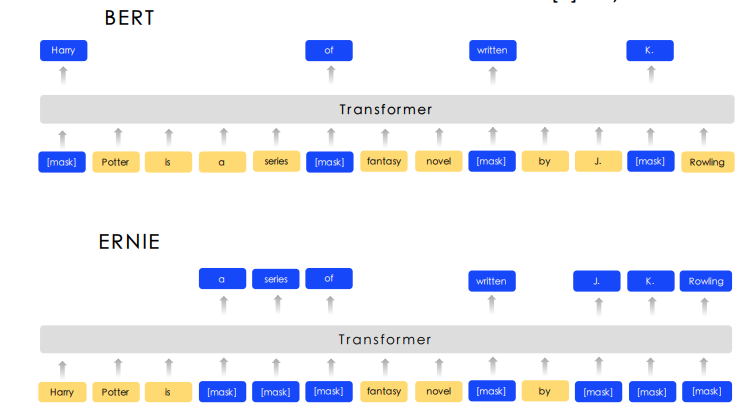### Recap: Techniques to add knowledge to LMS

1. Use pretrained entity embeddings
• Often too difficult to apply to existing archtectures to leverage KG pretraining
• Indirect way of incorporating knowledge and can be hard to interpret
• Can support some updating of factual knowledge and easier to interpret
• Tend to be more complex in implementation and require more memory
3. Modify the training data
• Requires no model changes or additional computation. May also be easiest to theoretically analyze! Active area of research
• Still open question if this is always as effective as model changes

### Evaluating knowledge in LMs

• Probe or Downstream tasks like QA

• LAnguage Model Analysis (LAMA) Probe
• How much relational (commonsense and factual) knowledge is already in off-the-shelf language models? - without any additional training or fine-tuning
• Manually constructed a set of cloze statements to assess a model’s ability to predict a missing token:
• The theory of relativity was developed by [MASK].
• The native language of Mammootty is [MASK].
• You are likely to find a overflow in a [MASK].
• Developing better prompts to query knowledge in LMs (Jiang et al TACL 2020)
• LMs may know the fact but failed on completion tasks like LAMA due to the query itself.
• the fact in pretraining data may have different contexts and sentence structure than the query.
• eg: The birth place of Barack Obama is Honolulu, Hawaii. vs Barack Obama was born in ___
• Generate more LAMA prompts by mining templates from Wikipedia and generating paraphrased prompts by using back-translation
• Ensemble prompts to increase diversity of contexts that fact can be seen in.
• Can we learn a prompt???

# Note 13 Model Analysis and Explanation

## Notes

### Motivating model analysis and explanation

• why do model predict what it predict?’
• understanding how far we can get incremental improvements on current methods is crucial to the eventual development of major improvements

### One model at multiple levels of abstraction

• At what level of abstraction you want to reason about your model?

• Model is a probability distribution and decision function

• Model is a sequence of vector representations in depth and time

• parameter weights, specific mechanisms like attention, dropout, etc